Published in Comput. Meth. Appl. Mech. Engng., Vol. 194 (21-24), pp. 2406-2443, 2005
doi: 10.1016/j.cma.2004.07.039

## ABSTRACT

A family of rotation-free three node triangular shell elements is presented. The simplest element of the family is based on an assumed constant curvature field expressed in terms of the nodal deflections of a patch of four elements and a constant membrane field computed from the standard linear interpolation of the displacements within each triangle. An enhanced version of the element is obtained by using a quadratic interpolation of the geometry in terms of the six patch nodes. This allows to compute an assumed linear membrane strain field which improves the in-plane behaviour of the original element. A simple and economic version of the element using a single integration point is presented. The efficiency of the different rotation-free shell triangles is demonstrated in many examples of application including linear and non linear analysis of shells under static and dynamic loads, the inflation and de-inflation of membranes and a sheet stamping problem.

## 1 INTRODUCTION

Triangular shell elements are very useful for the solution of large scale shell problems such as those occurring in many practical engineering situations. Typical examples are the analysis of shell roofs under static and dynamic loads, sheet stamping processes, vehicle dynamics and crash-worthiness situations. Many of these problems involve high geometrical and material non linearities and time changing frictional contact conditions. These difficulties are usually increased by the need of discretizing complex geometrical shapes. Here the use of shell triangles and non-structured meshes becomes a critical necessity. Despite recent advances in the field  there are not so many simple shell triangles which are capable of accurately modelling the deformation of a shell structure under arbitrary loading conditions.

A promising line to derive simple shell triangles is to use the nodal displacements as the only unknowns for describing the shell kinematics. This idea goes back to the original attempts to solve thin plate bending problems using finite difference schemes with the deflection as the only nodal variable .

In past years some authors have derived a number of thin plate and shell triangular elements free of rotational degrees of freedom (d.o.f.) based on Kirchhoff's theory . In essence all methods attempt to express the curvatures field over an element in terms of the displacements of a collection of nodes belonging to a patch of adjacent elements. Oñate and Cervera  proposed a general procedure of this kind combining finite element and finite volume concepts for deriving thin plate triangles and quadrilaterals with the deflection as the only nodal variable and presented a simple and competitive rotation-free three d.o.f. triangular element termed BPT (for Basic Plate Triangle). These ideas were extended and formalized in  to derive a number of rotation-free thin plate and shell triangles. The basic ingredients of the method are a mixed Hu-Washizu formulation, a standard discretization into three node triangles, a linear finite element interpolation of the displacement field within each triangle and a finite volume type approach for computing constant curvature and bending moment fields within appropriate non-overlapping control domains. The so called cell-centered and cell-vertex triangular domains yield different families of rotation-free plate and shell triangles. Both the BPT plate element and its extension to shell analysis (termed BST for Basic Shell Triangle) can be derived from the cell-centered formulation. Here the control domain is an individual triangle. The constant curvatures field within a triangle is computed in terms of the displacements of the six nodes belonging to the four elements patch formed by the chosen triangle and the three adjacent triangles. The cell-vertex approach yields a different family of rotation-free plate and shell triangles. Details of the derivation of both rotation-free triangular shell element families can be found in .

An extension of the BST element to the non linear analysis of shells was implemented in an explicit dynamic code by Oñate et al.  using an updated Lagrangian formulation and a hypo-elastic constitutive model. Excellent numerical results were obtained for non linear dynamics of shells involving frictional contact situations and sheet stamping problems [17,18,19,25].

A large strain formulation for the BST element using a total Lagrangian description was presented by Flores and Oñate . A recent extension of this formulation is based on a quadratic interpolation of the geometry of the patch formed by the BST element and the three adjacent triangles . This yields a linear displacement gradient field over the element from which linear membrane strains and constant curvatures can be computed within the BST element.

In this paper the formulation of the BST element is revisited using an assumed strain approach. The content of the paper is the following. First some basic concepts of the formulation of the original BST element using an assumed constant curvature field are given. Next, the basic equations of the non linear thin shell theory chosen based on a total Lagrangian description are presented. Then the non linear formulation of the BST element is presented. This is based on an assumed constant membrane field derived from the linear displacement interpolation and an assumed constant curvature field expressed in terms of the displacements of the nodes of the four element patch using a finite volume type approach. An enhanced version of the BST element is derived using an assumed linear field for the membrane strains and an assumed constant curvature field. Both assumed fields are obtained from the quadratic interpolation of the patch geometry following the ideas presented in . Details of the derivation of the tangent stiffness matrix needed for a quasi-static implicit solution are given for both the BST and EBST elements. An efficient version of the EBST element using one single quadrature point for integration of the tangent matrix is presented. An explicit scheme adequate for dynamic analysis is briefly described.

The efficiency and accuracy of the standard and enhanced versions of the BST element is validated in a number of examples of application including linear and non linear analysis of shells under static and dynamic loads, the inflation and de-inflation of membranes and a sheet stamping problem.

## 2 FORMULATION OF THE BASIC PLATE TRIANGLE USING AN ASSUMED CONSTANT CURVATURE FIELD

Let us consider a patch of four plate three node triangles (Figure 1). The nodes 1, 2, and 3 in the main central triangle (M) are marked with circles while the external nodes in the patch (nodes 4, 5 and 6) are marked with squares. Mid side points in the central triangle are also marked with smaller squares. Kirchhoff's thin plate theory will be assumed to hold. The deflection is linearly interpolated within each three node triangle in the standard finite element manner as

 $w=\sum _{i=1}^{3}L_{i}^{e}w_{i}^{e}$
(1)

where ${\textstyle L_{i}^{e}}$ are the linear shape functions (area coordinates) of the three node triangle, ${\textstyle w_{i}^{e}}$ are nodal deflections and superindex ${\textstyle e}$ denotes element values.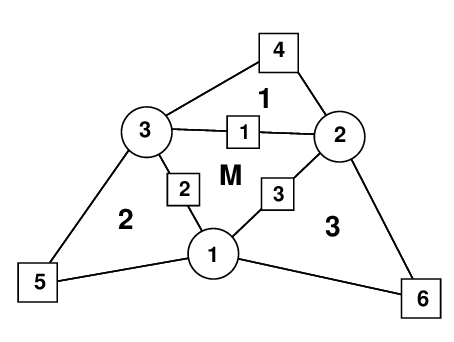Figure 1: Patch of three node triangular elements including the central triangle (M) and three adjacent triangles (1, 2 and 3)

The curvatures within the central triangle can be expressed in terms of a constant assumed curvatures field as

 ${\boldsymbol {\kappa }}=\left\{{\begin{array}{c}\kappa _{xx}\\\kappa _{yy}\\\kappa _{xy}\end{array}}\right\}={\hat {\boldsymbol {\kappa }}}$
(2)

where ${\textstyle {\boldsymbol {\kappa }}}$ is the curvature vector and ${\textstyle {\hat {\boldsymbol {\kappa }}}}$ is the assumed constant curvature field defined as

 ${\hat {\boldsymbol {\kappa }}}={\frac {1}{A_{M}}}\int \int _{A_{M}}\left[-{\frac {\partial ^{2}w}{\partial x^{2}}},-{\frac {\partial ^{2}w}{\partial y^{2}}},-2{\frac {\partial ^{2}w}{\partial x\partial y}}\right]^{T}dA$
(3)

where ${\textstyle A_{M}}$ is the area of the central triangle in Figure 1.

Integrating by parts Eq.(3) and substituting the resulting expression for ${\textstyle {\hat {\boldsymbol {\kappa }}}}$ into Eq.(2) gives the constant curvature field within the element as

 ${\boldsymbol {\kappa }}={\frac {1}{A_{M}}}\oint _{\Gamma _{M}}}\left[{\begin{array}{cc}-n_{x}&0\\0&-n_{y}\\-n_{y}&-n_{x}\end{array}}\right]\left\{{\begin{array}{c}{\dfrac {\partial w}{\partial x}}\\{\dfrac {\partial w}{\partial y}}\end{array}}\right\}d\Gamma$
(4)

where ${\textstyle \Gamma _{M}}$ is the boundary of the central triangle and ${\textstyle \mathbf {n} =\left(n_{x},n_{y}\right)}$ is the boundary normal. Equation (4) defines the assumed constant curvature field within the central triangle in terms of the deflection gradient along the sides of the triangle. Equation (4) can be found to be equivalent to the standard conservation laws used in finite volume procedures as described in [27,28].

The computation of the line integral in Eq.(4) poses a difficulty as the deflection gradient is discontinuous along the element sides. A simple method to overcome this problem is to compute the deflection gradient at the element sides as the average values of the gradient contributed by the two triangles sharing the side [20,28]. Following this idea the constant curvature field with the element is computed as

 ${\boldsymbol {\kappa }}={\frac {1}{A_{M}}}\sum _{j=1}^{3}{\frac {l_{j}^{M}}{2}}\left[{\begin{array}{cc}-n_{x}^{j}&0\\0&-n_{y}^{j}\\-n_{y}^{j}&-n_{x}^{j}\end{array}}\right]^{M}\left[{\nabla }L_{i}^{M}w_{i}^{M}+{\nabla }L_{i}^{j}w_{i}^{j}\right]$ $=\sum _{j=1}^{3}\left[{\begin{array}{cc}L_{j,x}^{M}&0\\0&L_{j,y}^{M}\\L_{j,y}^{M}&L_{j,x}^{M}\end{array}}\right]\left[{\nabla }L_{i}^{M}w_{i}^{M}+{\nabla }L_{i}^{j}w_{i}^{j}\right]=\mathbf {B} _{b}\mathbf {w} ^{p}$
(5)

where ${\textstyle \mathbf {w} ^{p}=[w_{1},w_{2},w_{3},w_{4},w_{5},w_{6}]^{T}}$ is the deflection vector of the six nodes in the patch. In Eq.(5) the sum extends over the three sides of the central element ${\textstyle M}$, ${\textstyle l_{j}^{M}}$ are the lengths of the element sides and superindexes ${\textstyle M}$ and ${\textstyle j}$ refer to the central triangle and to each of the adjacent elements, respectively. The standard sum convention for repeated indexes is used. Note that triangular area coordinates satisfy

 $\nabla L_{i}^{M}=\left[{\begin{array}{c}L_{i,x}^{M}\\L_{i,y}^{M}\end{array}}\right]=-{\frac {l_{i}^{M}}{2A_{M}}}\left[{\begin{array}{c}n_{x}^{i}\\n_{y}^{i}\end{array}}\right]$
(6)

Note also that the constant curvature field is expressed in terms of the six nodes of the four element patch linked to the element ${\textstyle M}$. The expression of the ${\textstyle 3\times {6}}$ ${\textstyle \mathbf {B} _{b}}$ matrix can be found in [14,20].

The virtual work expression is written as

 $\int \int _{A}\delta {\boldsymbol {\kappa }}^{T}\mathbf {m} \,dA=\int \int _{A}\delta w\,q\,dA$
(7)

where m is the bending moment field related to the curvatures by the standard constitutive equations

 $\mathbf {m} =[M_{xx},M_{yy},M_{xy}]^{T}=\mathbf {D} _{b}{\boldsymbol {\kappa }}\quad ,\quad \mathbf {D} _{b}={\frac {h^{3}}{12}}{\frac {E}{(1-\nu ^{2})}}\left[{\begin{array}{ccc}1&\nu &0\\\nu &1&0\\0&0&{\frac {1-\nu }{2}}\end{array}}\right]={\frac {h^{3}}{12}}\mathbf {D}$
(8)

In Eqs.(7) and (8) ${\textstyle h}$ is the plate thickness, ${\textstyle E}$ is the Young's modulus, ${\textstyle \nu }$ is the Poisson's ratio, ${\textstyle \delta {\boldsymbol {\kappa }}}$ and ${\textstyle \delta w}$ are the virtual curvatures and the virtual deflection, respectively, and ${\textstyle q}$ is a distributed vertical load.

Substituting the approximation for the vertical deflection and the assumed constant curvature field into (7) leads to the standard linear system of equations

 $\mathbf {K} \mathbf {w} =\mathbf {f}$
(9)

where the stiffness matrix ${\textstyle \mathbf {K} }$ and the equivalent nodal force ${\textstyle \mathbf {f} }$ can be found by assembly of the element contributions given by

 $\mathbf {K} ^{e}=\int \int _{A^{e}}\mathbf {B} _{b}^{T}\mathbf {D} _{b}\mathbf {B} _{b}dA$
(10)
 $\mathbf {f} ^{e}=\int \int _{A^{e}}q\left\{{\begin{array}{c}L_{1}^{e}\\L_{2}^{e}\\L_{3}^{e}\end{array}}\right\}dA$
(11)

Note that ${\textstyle \mathbf {K} ^{e}}$ is a ${\textstyle 6\times {6}}$ matrix, whereas ${\textstyle \mathbf {f} ^{e}}$ has the same structure than for the standard linear triangle. The explicit form of ${\textstyle \mathbf {K} ^{e}}$ and ${\textstyle \mathbf {f} ^{e}}$ can be found in .

The resulting Basic Plate Triangle (BPT) has one degree of freedom per node and a wider bandwidth than the standard three node triangles as each triangular element is linked to its three neighbours through Eq.(5).

Examples of the good performance of the BPT element for analysis of thin plates can be found in [14,20]. The extension of the BPT element to the analysis of shells yields the Basic Shell Triangle (BST) . Different applications of the BST element to linear and non linear analysis of shells are reported in [14,17–20,23,25,26].

The ideas used to derive the BPT element will now be extended to derive two families of Basic Shell Triangles using a total Lagrangian description.

## 3 BASIC THIN SHELL EQUATIONS USING A TOTAL LAGRANGIAN FORMULATION

### 3.1 Shell kinematics

A summary of the most relevant hypothesis related to the kinematic behaviour of a thin shell are presented. Further details may be found in the wide literature dedicated to this field [8,9].

Consider a shell with undeformed middle surface occupying the domain ${\textstyle \Omega ^{0}}$ in ${\textstyle R^{3}}$ with a boundary ${\textstyle \Gamma ^{0}}$. At each point of the middle surface a thickness ${\textstyle h^{0}}$ is defined. The positions ${\textstyle \mathbf {x} ^{0}}$ and ${\textstyle \mathbf {x} }$ of a point in the undeformed and the deformed configurations can be respectively written as a function of the coordinates of the middle surface ${\textstyle {\boldsymbol {\varphi }}}$ and the normal ${\textstyle \mathbf {t} _{3}}$ at the point as

 $\mathbf {x} ^{0}\left(\xi _{1},\xi _{2},\zeta \right)={\boldsymbol {\varphi }}^{0}\left(\xi _{1},\xi _{2}\right)+\lambda \mathbf {t} _{3}^{0}$ (12) $\mathbf {x} \left(\xi _{1},\xi _{2},\zeta \right)={\boldsymbol {\varphi }}\left(\xi _{1},\xi _{2}\right)+\zeta \lambda \mathbf {t} _{3}$ (13)

where ${\textstyle \xi _{1},\xi _{2}}$ are arc-length curvilinear principal coordinates defined over the middle surface of the shell and ${\textstyle \zeta }$ is the distance from the point to the middle surface in the undeformed configuration. The product ${\textstyle \zeta \lambda }$ is the distance from the point to the middle surface measured on the deformed configuration. The parameter ${\textstyle \lambda }$ relates the thickness at the present and initial configurations as:

 $\lambda ={\frac {h}{h^{0}}}$
(14)

This approach implies a constant strain in the normal direction. Parameter ${\textstyle \lambda }$ will not be considered as an independent variable and will be computed from purely geometrical considerations (isochoric behaviour) via a staggered iterative update. Besides this, the usual plane stress condition of thin shell theory will be adopted.

A convective system is computed at each point as

 $\mathbf {g} _{i}\left(\mathbf {\xi } \right)={\frac {\partial \mathbf {x} }{\partial \xi _{i}}}\qquad i=1,2,3$
(15)
 $\mathbf {g} _{\alpha }\left(\mathbf {\xi } \right)={\frac {\partial \left(\mathbf {\boldsymbol {\varphi }} \left(\xi _{1},\xi _{2}\right)+\zeta \lambda \mathbf {t} _{3}\right)}{\partial \xi _{\alpha }}}={\boldsymbol {\varphi }}_{^{\prime }\alpha }+\zeta \left(\lambda \mathbf {t} _{3}\right)_{^{\prime }\alpha }\quad \alpha =1,2$ (16) $\mathbf {g} _{3}\left(\mathbf {\xi } \right)={\frac {\partial \left(\mathbf {\boldsymbol {\varphi }} \left(\xi _{1},\xi _{2}\right)+\zeta \lambda \mathbf {t} _{3}\right)}{\partial \zeta }}=\lambda \mathbf {t} _{3}$ (17)

This can be particularized for the points on the middle surface as

 $\mathbf {a} _{\alpha }=\mathbf {g} _{\alpha }\left(\zeta =0\right)={\boldsymbol {\varphi }}_{^{\prime }\alpha }$ (18) $\mathbf {a} _{3}=\mathbf {g} _{3}\left(\zeta =0\right)=\lambda \mathbf {t} _{3}$ (19)

The covariant (first fundamental form) metric tensor of the middle surface is

 $a_{\alpha \beta }=\mathbf {a} _{\alpha }\cdot \mathbf {a} _{\beta }={\boldsymbol {\varphi }}_{^{\prime }\alpha }\cdot {\boldsymbol {\varphi }}_{^{\prime }\beta }$
(20)

The Green-Lagrange strain vector of the middle surface points (membrane strains) is defined as

 ${\boldsymbol {\varepsilon }}_{m}=[\varepsilon _{m_{11}},\varepsilon _{m_{12}},\varepsilon _{m_{12}}]^{T}$
(21)

with

 $\varepsilon _{m_{ij}}={\frac {1}{2}}(a_{ij}-a_{ij}^{0})$
(22)

The curvatures (second fundamental form) of the middle surface are obtained by

 $\kappa _{\alpha \beta }={\frac {1}{2}}\left({\boldsymbol {\varphi }}_{^{\prime }\alpha }\cdot \mathbf {t} _{3^{\prime }\beta }+{\boldsymbol {\varphi }}_{^{\prime }\beta }\cdot \mathbf {t} _{3^{\prime }\alpha }\right)=-\mathbf {t} _{3}\cdot {\boldsymbol {\varphi }}_{{\prime }\alpha \beta }\quad ,\quad \alpha ,\beta =1,2$
(23)

 $\mathbf {F=} [{\boldsymbol {x}}_{{\prime }1},{\boldsymbol {x}}_{{\prime }2},{\boldsymbol {x}}_{{\prime }3}]=\left[{\begin{array}{ccc}{\boldsymbol {\varphi }}_{^{\prime }1}+\zeta \left(\lambda \mathbf {t} _{3}\right)_{^{\prime }1}&{\boldsymbol {\varphi }}_{^{\prime }2}+\zeta \left(\lambda \mathbf {t} _{3}\right)_{^{\prime }2}&\lambda \mathbf {t} _{3}\end{array}}\right]$
(24)

The product ${\textstyle \mathbf {F} ^{T}\mathbf {F=U} ^{2}=\mathbf {C} }$ (where ${\textstyle \mathbf {U} }$ is the right stretch tensor, and ${\textstyle \mathbf {C} }$ the right Cauchy-Green deformation tensor) can be written as

 $\mathbf {U} ^{2}=\left[{\begin{array}{ccc}a_{11}+2\kappa _{11}\zeta \lambda &a_{12}+2\kappa _{12}\zeta \lambda &0\\a_{12}+2\kappa _{12}\zeta \lambda &a_{22}+2\kappa _{22}\zeta \lambda &0\\0&0&\lambda ^{2}\end{array}}\right]$
(25)

In the derivation of expression (25) the derivatives of the thickness ratio ${\textstyle \lambda _{^{\prime }a}}$ and the terms associated to ${\textstyle \zeta ^{2}}$ have been neglected.

Equation (25) shows that ${\textstyle \mathbf {U} ^{2}}$ is not a unit tensor at the original configuration for curved surfaces (${\textstyle \kappa _{ij}^{0}\neq {0}}$). The changes of curvature of the middle surface are computed by

 $\chi _{ij}=\kappa _{ij}-\kappa _{ij}^{0}$
(26)

Note that ${\textstyle \delta \chi _{ij}=\delta \kappa _{ij}}$.

For computational convenience the following approximate expression (which is exact for initially flat surfaces) will be adopted

 $\mathbf {U} ^{2}=\left[{\begin{array}{ccc}a_{11}+2\chi _{11}\zeta \lambda &a_{12}+2\chi _{12}\zeta \lambda &0\\a_{12}+2\chi _{12}\zeta \lambda &a_{22}+2\chi _{22}\zeta \lambda &0\\0&0&\lambda ^{2}\end{array}}\right]$
(27)

This expression is useful to compute different Lagrangian strain measures. An advantage of these measures is that they are associated to material fibres, what makes it easy to take into account material anisotropy. It is also useful to compute the eigen decomposition of ${\textstyle \mathbf {U} }$ as

 $\mathbf {U=} \sum _{\alpha =1}^{3}\lambda _{\alpha }\mathbf {r} _{\alpha }\otimes \mathbf {r} _{\alpha }$
(28)

where ${\textstyle \lambda _{\alpha }}$ and ${\textstyle \mathbf {r} _{\alpha }}$ are the eigenvalues and eigenvectors of ${\textstyle \mathbf {U} }$.

The resultant stresses (axial forces and moments) are obtained by integrating across the original thickness the second Piola-Kirchhoff stress vector ${\textstyle {\boldsymbol {\sigma }}}$ using the actual distance to the middle surface for evaluating the bending moments. This gives

 ${\boldsymbol {\sigma }}_{m}\equiv \lbrack N_{11},N_{22},N_{12}]^{T}=\int _{h^{0}}{\boldsymbol {\sigma }}d\zeta$
(29)

 ${\boldsymbol {\sigma }}_{b}\equiv \lbrack M_{11},M_{22},M_{12}]^{T}=\int _{h^{0}}{\boldsymbol {\sigma }}\lambda \zeta d\zeta$
(30)

With these values the virtual work can be written as

 $\int \int _{A^{0}}\left[\delta {\boldsymbol {\varepsilon }}_{m}^{T}{\boldsymbol {\sigma }}_{m}+\delta {\boldsymbol {\kappa }}^{T}{\boldsymbol {\sigma }}_{b}\right]dA=\int \int _{A^{0}}\delta \mathbf {u} ^{T}\mathbf {t} dA$
(31)

where ${\textstyle \delta \mathbf {u} }$ are virtual displacements, ${\textstyle \delta {\boldsymbol {\varepsilon }}_{m}}$ is the virtual Green-Lagrange membrane strain vector, ${\textstyle \delta {\boldsymbol {\kappa }}}$ are the virtual curvatures and ${\textstyle \mathbf {t} }$ are the surface loads. Other load types can be easily included into (31).

### 3.2 Constitutive models

In order to treat plasticity at finite strains an adequate stress-strain pair must be used. The Hencky measures will be adopted here. The (logarithmic) strains are defined as

 $\mathbf {E} _{\ln }\mathbf {=} \left[{\begin{array}{ccc}\varepsilon _{11}&\varepsilon _{21}&0\\\varepsilon _{12}&\varepsilon _{22}&0\\0&0&\varepsilon _{33}\end{array}}\right]=\sum _{\alpha =1}^{3}\ln \left(\lambda _{\alpha }\right)\mathbf {r} _{\alpha }\otimes \mathbf {r} _{\alpha }$
(32)

Two types of material models are considered here: an elastic-plastic material associated to thin rolled metal sheets and a hyper-elastic material for rubbers.

In the case of metals, where the elastic strains are small, the use of a logarithmic strain measure reasonably allows to adopt an additive decomposition of elastic and plastic components as

 $\mathbf {E} _{\ln }\mathbf {=E} _{\ln }^{e}+\mathbf {E} _{\ln }^{p}$
(33)

A constant linear relationship between the (plane) Hencky stresses and the logarithmic elastic strains is adopted giving

 $\mathbf {T} =\mathbf {D} \mathbf {E} _{\ln }^{e}$
(34)

These constitutive equations are integrated using a standard return algorithm. The following Mises-Hill  yield function with non-linear isotropic hardening is chosen

 $\left(G+H\right)\;T_{11}^{2}+\left(F+H\right)\;T_{22}^{2}-2H\;T_{11}T_{22}+2N\;T_{12}^{2}=\sigma _{0}\left(e_{0}+e^{p}\right)^{n}$
(35)

where ${\textstyle F,G,H}$ and ${\textstyle N}$ define the non-isotropic shape of the yield surface and the parameters ${\textstyle \sigma _{0}}$, ${\textstyle e_{0}}$ and ${\textstyle n}$ define its size as a function of the effective plastic strain ${\textstyle e^{p}}$.

The simple Mises-Hill yield function allows, as a first approximation, to treat rolled thin metal sheets with planar and transversal anisotropy.

For the case of rubbers, the Ogden  model extended to the compressible range is considered. The material behaviour is characterized by the strain energy density per unit undeformed volume defined as

 $\psi ={\frac {K}{2}}\left(\ln J\right)^{2}+\sum _{p=1}^{N}{\frac {\mu _{p}}{\alpha _{p}}}\left[J^{-{\frac {\alpha _{p}}{3}}}\left(\sum _{i=1}^{3}\lambda _{i}^{\alpha _{p}-1}\right)-3\right]$
(36)

where ${\textstyle K}$ is the bulk modulus of the material, ${\textstyle J}$ is the determinant of ${\textstyle \mathbf {U} }$, ${\textstyle N}$, ${\textstyle \mu _{i}}$ and ${\textstyle \alpha _{i}}$ are material parameters, ${\textstyle \mu _{i}\,,\,\alpha _{i}}$ are real numbers such that ${\textstyle \mu _{i}\alpha _{i}>0}$ ${\textstyle (\forall i=1,N)}$ and ${\textstyle N}$ is a positive integer.

The stress measures associated to the principal logarithmic strains are denoted by ${\textstyle \beta _{i}}$. They can be computed noting that

 $\beta _{i}={\frac {\partial \psi \left(\lambda _{\alpha }\right)}{\partial \left(\ln \lambda _{i}\right)}}=K\left(\ln J\right)+\lambda _{i}\sum _{p=1}^{N}\mu _{p}J^{-{\frac {\alpha _{p}}{3}}}\left(\lambda _{i}^{\alpha _{p}-1}-{\frac {1}{3}}{\frac {1}{\lambda _{i}}}\sum _{j=1}^{3}\lambda _{j}^{\alpha _{p}}\right)$
(37)

we define now

 $a^{p}=\sum _{j=1}^{3}\lambda _{j}^{\alpha _{p}}$
(38)

which gives

 $\beta _{i}=K\left(\ln J\right)+\sum _{p=1}^{N}\mu _{p}J^{-{\frac {\alpha _{p}}{3}}}\left(\lambda _{i}^{\alpha _{p}}-{\frac {1}{3}}a_{p}\right)$
(39)

The values of ${\textstyle \beta _{i}}$, expressed in the principal strains directions, allow to evaluate the Hencky stresses in the convective coordinate system as

 $\mathbf {T} =\sum _{i=1}^{3}\beta _{i}\;\mathbf {r} _{i}\otimes \mathbf {r} _{i}$
(40)

The Hencky stress tensor ${\textstyle \mathbf {T} }$ can be easily particularized for the plane stress case.

We define the rotated Hencky and second Piola-Kirchhoff stress tensors as

 $\mathbf {T} _{L}=\mathbf {R} _{L}^{T}\;\mathbf {T\;R} _{L}$ (41) $\mathbf {S} _{L}=\mathbf {R} _{L}^{T}\;\mathbf {S\;R} _{L}$ (42)

where ${\textstyle \mathbf {R} _{L}}$ is the rotation tensor obtained from the eigenvectors of ${\textstyle \mathbf {U} }$ given by

 $\mathbf {R} _{L}=\left[{\begin{array}{ccc}\mathbf {r} _{1}\quad ,&\mathbf {r} _{2}\quad ,&\mathbf {r} _{3}\end{array}}\right]$
(43)

The relationship between the rotated Hencky and Piola-Kirchhoff stresses is ${\textstyle \left(\alpha ,\beta =1,2\right)}$

 $\left[S_{L}\right]_{\alpha \alpha }={\frac {1}{\lambda _{\alpha }^{2}}}\left[T_{L}\right]_{\alpha \alpha }$ $\left[S_{L}\right]_{\alpha \beta }={\frac {\ln \left(\lambda _{\alpha }/\lambda _{\beta }\right)}{{\frac {1}{2}}\left(\lambda _{\alpha }^{2}-\lambda _{\beta }^{2}\right)}}\left[T_{L}\right]_{\alpha \beta }$
(44)

The second Piola-Kirchhoff stress tensor can be computed by

 $\mathbf {S=} \sum _{\alpha =1}^{2}\sum _{\beta =1}^{2}\left[S_{L}\right]_{\alpha \beta }\mathbf {r} _{\alpha }\otimes \mathbf {r} _{\beta }$
(45)

The second Piola-Kirchhoff stress vector ${\textstyle {\boldsymbol {\sigma }}}$ of Eqs.(2930) can be readily extracted from the ${\textstyle \mathbf {S} }$ tensor.

## 4 TOTAL LAGRANGIAN FORMULATION OF THE BASIC SHELL TRIANGLE

### 4.1 Definition of the element geometry and discretization of the displacement field

The rotation-free BST element has three nodes with three displacement degrees of freedom at each node. As for the BPT element a patch is defined by the central triangle and the three adjacent elements (Figure 1). This four elements patch helps to define the curvature field within the central triangle (the BST element) in terms of the displacements of the six patch nodes.

The node-ordering in the patch is the following (see Figure 1)

• The nodes in the main element (M) are numbered locally as 1, 2 and 3. They are defined counter-clockwise around the positive normal.
• The sides in the main element are numbered locally as 1, 2, and 3. They are defined by the local node opposite to the side.
• The adjacent elements (which are part of the patch) are numbered with the number associated to the common side.
• The extra nodes of the patch are numbered locally as 4, 5 and 6, corresponding to nodes on adjacent elements opposite to sides 1, 2 and 3 respectively.
• The connectivities in the adjacent elements are defined beginning with the extra node as shown in Table 1.

 Element N1 N2 N3 M 1 2 3 1 4 3 2 2 5 1 3 3 6 2 1

The following local Cartesian coordinate system is defined for the patch. In the main element the unit vector ${\textstyle \mathbf {t} _{1}}$(associated to the local coordinate ${\textstyle \xi _{1}}$) is directed along side 3 (from node 1 to node 2), ${\textstyle \mathbf {t} _{3}}$ (associated to the coordinate ${\textstyle \zeta }$) is the unit normal to the plane, and finally ${\textstyle \mathbf {t} _{2}=\mathbf {t} _{3}\times \mathbf {t} _{1}}$ (associated to the coordinate ${\textstyle \xi _{2}}$).

The coordinates and the displacements are linearly interpolated within each three node triangle in the mesh in the standard manner, i.e.

 ${\boldsymbol {\varphi }}=\sum \limits _{i=1}^{3}L_{i}^{e}{\boldsymbol {\varphi }}_{i}=\sum \limits _{i=1}^{3}L_{i}^{e}({\boldsymbol {\varphi }}_{i}^{0}+\mathbf {u} _{i})$
(46)
 $\mathbf {u} =\left\{{\begin{array}{c}u_{1}\\u_{2}\\u_{3}\end{array}}\right\}=\sum \limits _{i=1}^{3}L_{i}^{e}\mathbf {u} _{i}\quad ,\quad \mathbf {u} _{i}=\left\{{\begin{array}{c}u_{1}\\u_{2}\\u_{3}\end{array}}\right\}_{i}$
(47)

In above ${\textstyle {\boldsymbol {\varphi }}_{i}}$ and ${\textstyle \mathbf {u} _{i}}$ contain respectively the three coordinates and the three displacements of node ${\textstyle i}$.

### 4.2 Computation of the membrane strains

The Green-Lagrange membrane strains are expressed by substituting the linear displacement interpolation into Eq.(22). This gives

 ${\boldsymbol {\varepsilon }}_{m}={\frac {1}{2}}\left[{\begin{array}{c}{\boldsymbol {\varphi }}_{^{\prime }1}\cdot {\boldsymbol {\varphi }}_{^{\prime }1}-1\\{\boldsymbol {\varphi }}_{^{\prime }2}\cdot {\boldsymbol {\varphi }}_{^{\prime }2}-1\\2{\boldsymbol {\varphi }}_{^{\prime }1}\cdot {\boldsymbol {\varphi }}_{^{\prime }2}\end{array}}\right]$
(48)

The membrane strain field is constant within each triangle similarly as in the standard CST element. The variation of the membrane strains is obtained by

 $\delta {\boldsymbol {\varepsilon }}_{m}=\mathbf {B} _{m}\delta \mathbf {a} ^{e}$
(49)

with

 $\mathbf {B} _{m}=[\mathbf {B} _{m_{1}},\mathbf {B} _{m_{2}},\mathbf {B} _{m_{3}}]\quad ,\quad \mathbf {a} ^{e}=\left\{{\begin{array}{c}\mathbf {u} _{1}\\\mathbf {u} _{2}\\\mathbf {u} _{3}\end{array}}\right\}$
(50)

and

 ${\begin{array}{c}\\\mathbf {B} _{m_{i}}\\3\times {3}\end{array}}=\left[{\begin{array}{c}L_{i,1}^{M}{\boldsymbol {\varphi }}_{^{\prime }1}^{T}\\L_{i,2}^{M}{\boldsymbol {\varphi }}_{^{\prime }2}^{T}\\L_{i,1}^{M}{\boldsymbol {\varphi }}_{^{\prime }2}^{T}+L_{i,2}^{M}{\boldsymbol {\varphi }}_{^{\prime }1}^{T}\end{array}}\right]$
(51)

### 4.3 Computation of bending strains (curvatures)

We will assume the following constant curvature field within each element

 $\kappa _{\alpha \beta }={\hat {\kappa }}_{\alpha \beta }$
(52)

where ${\textstyle {\hat {\kappa }}_{\alpha \beta }}$ is the assumed constant curvature field defined by

 ${\hat {\kappa }}_{\alpha \beta }=-{\frac {1}{A_{M}^{0}}}\int _{A_{M}^{0}}\mathbf {t} _{3}\cdot {\boldsymbol {\varphi }}_{^{\prime }\beta \alpha }\;dA^{0}$
(53)

where ${\textstyle A_{M}^{0}}$ is the area (in the original configuration) of the central element in the patch.

Substituting Eq.(53) into (52) and integrating by parts the area integral gives the curvature vector within the element in terms of the following line integral

 ${\boldsymbol {\kappa }}=\left\{{\begin{array}{c}\kappa _{11}\\\kappa _{22}\\2\kappa _{12}\end{array}}\right\}={\frac {1}{A_{M}^{0}}}\oint _{\Gamma _{M}^{0}}}\left[{\begin{array}{cc}-n_{1}&0\\0&-n_{2}\\-n_{2}&-n_{1}\end{array}}\right]\left[{\begin{array}{c}\mathbf {t} _{3}\cdot {\boldsymbol {\varphi }}_{^{\prime }1}\\\mathbf {t} _{3}\cdot {\boldsymbol {\varphi }}_{^{\prime }2}\end{array}}\right]d\Gamma$
(54)

where ${\textstyle n_{i}}$ are the components (in the local system) of the normals to the element sides in the initial configuration ${\textstyle \Gamma _{M}^{0}}$.

For the definition of the normal vector ${\textstyle \mathbf {t} _{3}}$, the linear interpolation over the central element is used. In this case the tangent plane components are

 ${\boldsymbol {\varphi }}_{^{\prime }\alpha }=\sum _{i=1}^{3}L_{i,\alpha }^{M}{\boldsymbol {\varphi }}_{i}\quad ,\quad \alpha =1,2$
(55)

 $\mathbf {t} _{3}={\frac {{\boldsymbol {\varphi }}_{\prime {1}}\times {\boldsymbol {\varphi }}_{\prime {2}}}{\left\vert {\boldsymbol {\varphi }}_{\prime {1}}\times {\boldsymbol {\varphi }}_{\prime {2}}\right\vert }}=\lambda \;{\boldsymbol {\varphi }}_{1}\times {\boldsymbol {\varphi }}_{2}$
(56)

From these expressions it is also possible to compute in the original configuration the element area ${\textstyle A_{M}^{0}}$, the outer normals ${\textstyle \left(n_{1},n_{2}\right)^{i}}$ at each side and the side lengths ${\textstyle l_{i}^{M}}$. Equation (56) also allows to evaluate the thickness ratio ${\textstyle \lambda }$ in the deformed configuration and the actual normal ${\textstyle \mathbf {t} _{3}}$.

In order to compute the line integral of equation (54) the averaging procedure described in Section 2 is used. Hence along each side of the triangle the average value of ${\textstyle {\boldsymbol {\varphi }}_{^{\prime }\alpha }}$ between the main triangle and the adjacent one is taken leading to

 ${\boldsymbol {\kappa }}={\frac {1}{A_{M}^{0}}}\sum _{i=1}^{3}l_{i}^{M}\left[{\begin{array}{cc}-n_{1}^{i}&0\\0&-n_{2}^{i}\\-n_{2}^{i}&-n_{1}^{i}\end{array}}\right]\left[{\begin{array}{c}\mathbf {t} _{3}\cdot {\frac {1}{2}}\left(\mathbf {\boldsymbol {\varphi }} _{^{\prime }1}^{M}+\mathbf {\boldsymbol {\varphi }} _{^{\prime }1}^{i}\right)\\\mathbf {t} _{3}\cdot {\frac {1}{2}}\left(\mathbf {\boldsymbol {\varphi }} _{^{\prime }2}^{M}+\mathbf {\boldsymbol {\varphi }} _{^{\prime }2}^{i}\right)\end{array}}\right]$
(57)

where the sum extends over the three elements adjacent to the central triangle ${\textstyle M}$.

Noting that ${\textstyle \mathbf {t} _{3}\cdot \mathbf {\boldsymbol {\varphi }} _{^{\prime }\alpha }^{M}=0}$ in the main triangle and using (6) it can be found 

 ${\boldsymbol {\kappa }}=\sum _{i=1}^{3}\left[{\begin{array}{cc}L_{i,1}^{M}&0\\0&L_{i,2}^{M}\\L_{i,2}^{M}&L_{i,1}^{M}\end{array}}\right]\left[{\begin{array}{c}\mathbf {t} _{3}\cdot \mathbf {\boldsymbol {\varphi }} _{^{\prime }1}^{i}\\\mathbf {t} _{3}\cdot \mathbf {\boldsymbol {\varphi }} _{^{\prime }2}^{i}\end{array}}\right]$
(58)

This can be seen as the projection of the local derivatives of ${\textstyle {\boldsymbol {\varphi }}}$ in the adjacent triangles (${\textstyle \mathbf {\boldsymbol {\varphi }} _{^{\prime }\alpha }^{i}}$ where index ${\textstyle i}$ denotes values associated to the adjacent elements) over the normal to the main triangle ${\textstyle \mathbf {t} _{3}}$. As the triangles have a common side, ${\textstyle \mathbf {t} _{3}\cdot \mathbf {\boldsymbol {\varphi }} _{^{\prime }s}^{i}=0}$, where ${\textstyle \mathbf {\boldsymbol {\varphi }} _{^{\prime }s}^{i}}$ is the derivative of ${\textstyle {\boldsymbol {\varphi }}}$ along the side. Hence only the derivative of ${\textstyle {\boldsymbol {\varphi }}}$ along the side normal (${\textstyle \mathbf {\boldsymbol {\varphi }} _{^{\prime }n}^{i}}$) has non-zero component over ${\textstyle \mathbf {t} _{3}}$. This gives

 $\left[{\begin{array}{c}\mathbf {t} _{3}\cdot \mathbf {\boldsymbol {\varphi }} _{^{\prime }1}^{i}\\\mathbf {t} _{3}\cdot \mathbf {\boldsymbol {\varphi }} _{^{\prime }2}^{i}\end{array}}\right]=\left(\mathbf {t} _{3}\cdot \mathbf {\boldsymbol {\varphi }} _{^{\prime }n}^{i}\right)\mathbf {n} ^{i}$
(59)

An alternative form to express the curvatures, which is useful when their variations are needed, is to define the vectors

 $\mathbf {h} _{ij}=\sum _{k=1}^{3}{\frac {1}{2}}\left(L_{k,i}^{M}{\boldsymbol {\varphi }}_{^{\prime }j}^{k}+L_{k,j}^{M}{\boldsymbol {\varphi }}_{\prime i}^{k}\right)$
(60)

This gives

 $\kappa _{ij}=\mathbf {h} _{ij}\cdot \mathbf {t} _{3}$
(61)

The last expression allows to interpret the curvatures as the projections of the vectors ${\textstyle \mathbf {h} _{ij}}$ over the normal of the central element. The variation of the curvatures can be obtained as

 $\delta {\boldsymbol {\kappa }}=\sum _{i=1}^{3}\left\{\left[{\begin{array}{cc}L_{i,1}^{M}&0\\0&L_{i,2}^{M}\\L_{i,2}^{M}&L_{i,1}^{M}\end{array}}\right]\sum _{J=1}^{3}\left[{\begin{array}{c}L_{j,1}^{i}(\mathbf {t} _{3}\cdot \delta \mathbf {u} _{j}^{i})\\L_{j,2}^{i}(\mathbf {t} _{3}\cdot \delta \mathbf {u} _{j}^{i})\end{array}}\right]-2\left[{\begin{array}{c}(L_{i,1}^{M}\rho _{11}^{1}+L_{i,2}^{M}\rho _{11}^{2})\\(L_{i,1}^{M}\rho _{22}^{1}+L_{i,2}^{M}\rho _{22}^{2})\\(L_{i,1}^{M}\rho _{12}^{1}+L_{i,2}^{M}\rho _{12}^{2})\end{array}}\right](\mathbf {t} _{3}\cdot \delta \mathbf {u} _{i}^{M})\right\}$
(62)

where the projections of the vectors ${\textstyle \mathbf {h} _{ij}}$ over the contravariant base vectors ${\textstyle {\boldsymbol {\varphi }}^{\alpha }}$ have been included

 $\rho _{ij}^{\alpha }=\mathbf {h} _{ij}\cdot {\boldsymbol {\varphi }}^{\alpha }\quad ,\quad \alpha ,i,j=1,2$
(63)

with

 $\mathbf {\boldsymbol {\varphi }} ^{1}=\lambda \;\mathbf {\boldsymbol {\varphi }} _{^{\prime }2}\times \mathbf {t} _{3}$ (64) $\mathbf {\boldsymbol {\varphi }} ^{2}=-\lambda \;\mathbf {\boldsymbol {\varphi }} _{^{\prime }1}\times \mathbf {t} _{3}$ (65)

In above expressions superindexes in ${\textstyle L_{j}^{k}}$ and ${\textstyle \delta \mathbf {u} _{j}^{k}}$ refer to element numbers in the patch whereas subscripts denote node numbers of each element in the patch. As usual the superindex ${\textstyle M}$ denotes values in the central triangle (Figure 1). Note that as expected the curvatures (and their variations) in the central element are a function of the nodal displacements of the six nodes in the four elements patch. Note also the isochoric approach

 $\lambda ={\frac {h}{h^{0}}}={\frac {A_{M}^{0}}{A_{M}}}$
(66)

The derivation of Eq.(62) can be found in . This equation can be rewritten in the form

 $\delta {\boldsymbol {\kappa }}=\mathbf {B} _{b}\delta \mathbf {a} ^{p}$
(67)

where

 ${\begin{array}{c}\\\delta \mathbf {a} ^{p}\\18\times {1}\end{array}}=[\delta \mathbf {u} _{1}^{T},\delta \mathbf {u} _{2}^{T},\delta \mathbf {u} _{3}^{T},\delta \mathbf {u} _{4}^{T},\delta \mathbf {u} _{5}^{T},\delta \mathbf {u} _{6}^{T}]^{T}$
(68)

is the virtual displacement vector of the patch and

 $\mathbf {B} _{b}=[\mathbf {B} _{b1},\mathbf {B} _{b2},\cdots ,\mathbf {B} _{b6}]$
(69)

is the curvature matrix relating the virtual curvatures within the central element and the 18 virtual displacements of the six nodes in the patch.

The form of matrix ${\textstyle \mathbf {B} _{b}}$ is given in the Appendix.

## 5 ENHANCED BASIC SHELL TRIANGLE

An enhanced version of the BST element (termed EBST) has been recently proposed by Flores and Oñate . The main features of the element formulation are the following:

1. The geometry of the patch formed by the central element and the three adjacent elements is quadratically interpolated from the position of the six nodes in the patch.
2. The membrane strains are assumed to vary linearly within the central triangle and are expressed in terms of the (continuous) values of the deformation gradient at the mid side points of the triangle.
3. The assumed constant curvature field within the central triangle is obtained by expression (54) using now twice the values of the (continuous) deformation gradient at the mid side points.

Details of the derivation of the EBST element are given below.

### 5.1 Definition of the element geometry and computation of membrane strains

As mentioned above a quadratic approximation of the geometry of the four elements patch is chosen using the position of the six nodes in the patch. It is useful to define the patch in the isoparametric space using the nodal positions given in the Table 2 (see also Figure 2).

 1 2 3 4 5 6 ${\textstyle \xi }$ 0 1 0 1 -1 1 ${\textstyle \eta }$ 0 0 1 1 1 -1

The quadratic interpolation is defined by

 ${\boldsymbol {\varphi }}=\sum _{i=1}^{6}N_{i}{\boldsymbol {\varphi }}_{i}$
(70)

with (${\textstyle \zeta =1-\xi -\eta }$)

 ${\begin{array}{ccc}N_{1}=\zeta {+\xi }\eta &&N_{4}={\frac {\zeta }{2}}\left(\zeta {-1}\right)\\N_{2}=\xi {+\eta }\zeta &&N_{5}={\frac {\xi }{2}}\left(\xi {-1}\right)\\N_{3}=\eta {+\zeta }\xi &&N_{6}={\frac {\eta }{2}}\left(\eta {-1}\right)\end{array}}$
(71)

This interpolation allows to compute the displacement gradients at selected points in order to use an assumed strain approach. The computation of the gradients is performed at the mid side points of the central element of the patch denoted by ${\textstyle G_{1}}$, ${\textstyle G_{2}}$ and ${\textstyle G_{3}}$ in Figure 2. This choice has the following advantages.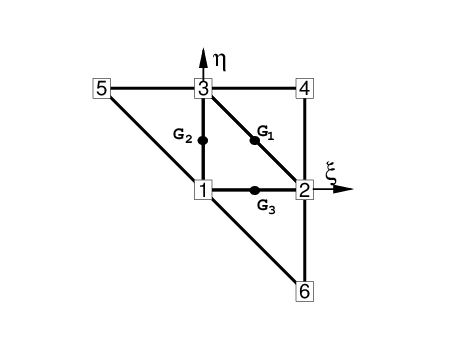Figure 2: Patch of elements in the isoparametric space.
• Gradients at the three mid side points depend only on the nodes belonging to the two elements adjacent to each side. This can be easily verified by sampling the derivatives of the shape functions at each mid-side point.
• When gradients are computed at the common mid-side point of two adjacent elements, the same values are obtained, as the coordinates of the same four points are used. This in practice means that the gradients at the mid-side points are independent of the element where they are computed. A side-oriented implementation of the finite element will therefore lead to a unique evaluation of the gradients per side.

The Cartesian derivatives of the shape functions are computed at the original configuration by the standard expression

 $\left[{\begin{array}{c}N_{i,1}\\N_{i,2}\end{array}}\right]=\mathbf {J} ^{-1}\left[{\begin{array}{c}N_{i,\xi }\\N_{i,\eta }\end{array}}\right]$
(72)

where the Jacobian matrix at the original configuration is

 $\mathbf {J=} \left[{\begin{array}{cc}\mathbf {\boldsymbol {\varphi }} _{^{\prime }\xi }^{0}\cdot \mathbf {t} _{1}&\mathbf {\boldsymbol {\varphi }} _{^{\prime }\eta }^{0}\cdot \mathbf {t} _{1}\\\mathbf {\boldsymbol {\varphi }} _{^{\prime }\xi }^{0}\cdot \mathbf {t} _{2}&\mathbf {\boldsymbol {\varphi }} _{^{\prime }\eta }^{0}\cdot \mathbf {t} _{2}\end{array}}\right]$
(73)

The deformation gradients on the middle surface, associated to an arbitrary spatial Cartesian system and to the material cartesian system defined on the middle surface are related by

 $\left[{\boldsymbol {\varphi }}_{^{\prime }1},\mathbf {\boldsymbol {\varphi }} _{^{\prime }2}\right]=\left[\mathbf {\boldsymbol {\varphi }} _{^{\prime }\xi },\mathbf {\boldsymbol {\varphi }} _{^{\prime }\eta }\right]\mathbf {J} ^{-1}$
(74)

The membrane strains within the central triangle are now obtained using a linear assumed strain field ${\textstyle {\hat {\boldsymbol {\varepsilon }}}_{m}}$. If, for example, Green Lagrange strains are used, i.e.

 ${\boldsymbol {\varepsilon }}_{m}={\hat {\boldsymbol {\varepsilon }}}_{m}$
(75)

with

 ${\hat {\boldsymbol {\varepsilon }}}_{m}=(1-2\zeta ){\boldsymbol {\varepsilon }}_{m}^{1}+(1-2\xi ){\boldsymbol {\varepsilon }}_{m}^{2}+(1-2\eta ){\boldsymbol {\varepsilon }}_{m}^{3}=\sum _{i=1}^{3}{\bar {N}}_{i}{\boldsymbol {\varepsilon }}_{m}^{i}$
(76)

where ${\textstyle {\boldsymbol {\varepsilon }}_{m}^{i}}$ are the membrane strains computed at the three mid side points ${\textstyle G_{i}}$ (${\textstyle i=1,2,3}$ see Figure 2). In Eq.(76) ${\textstyle {\bar {N}}_{1}=(1-2\zeta )}$, etc.

The gradient at each mid side point is computed from the quadratic interpolation (70):

 $\left({\boldsymbol {\varphi }}_{^{\prime }\alpha }\right)_{G_{i}}={\boldsymbol {\varphi }}_{^{\prime }\alpha }^{i}=\left[\sum _{j=1}^{3}N_{j,\alpha }^{i}{\boldsymbol {\varphi }}_{j}\right]+N_{i+3,\alpha }^{i}{\boldsymbol {\varphi }}_{i+3}\quad ,\quad \alpha =1,2\quad ,\quad i=1,2,3$
(77)

Substituting Eq.(22) into (76) and using Eq.(20) gives the membrane strain vector as

 ${\boldsymbol {\varepsilon }}_{m}=\sum _{i=1}^{3}{\frac {1}{2}}{\bar {N}}_{i}\left\{{\begin{array}{c}{\boldsymbol {\varphi }}_{^{\prime }1}^{i}\cdot \mathbf {\boldsymbol {\varphi }} _{^{\prime }1}^{i}-1\\{\boldsymbol {\varphi }}_{^{\prime }2}^{i}\cdot \mathbf {\boldsymbol {\varphi }} _{^{\prime }2}^{i}-1\\2{\boldsymbol {\varphi }}_{^{\prime }1}^{i}\cdot \mathbf {\boldsymbol {\varphi }} _{^{\prime }2}^{i}\end{array}}\right\}$
(78)

and the virtual membrane strains as

 $\delta {\boldsymbol {\varepsilon }}_{m}=\sum _{i=1}^{3}{\bar {N}}_{i}\left\{{\begin{array}{c}{\boldsymbol {\varphi }}_{^{\prime }1}^{i}\cdot \delta \mathbf {\boldsymbol {\varphi }} _{^{\prime }1}^{i}\\{\boldsymbol {\varphi }}_{2}^{i}\cdot \delta \mathbf {\boldsymbol {\varphi }} _{^{\prime }2}^{i}\\\delta {\boldsymbol {\varphi }}_{^{\prime }1}^{i}\cdot \mathbf {\boldsymbol {\varphi }} _{^{\prime }2}^{i}+{\boldsymbol {\varphi }}_{^{\prime }1}^{i}\cdot \delta \mathbf {\boldsymbol {\varphi }} _{2}^{i}\end{array}}\right\}$
(79)

We note that the gradient at each mid side point ${\textstyle G_{i}}$ depends only on the coordinates of the three nodes of the central triangle and on those of an additional node in the patch, associated to the side ${\textstyle i}$ where the gradient is computed.

Combining Eqs.(79) and (77) gives

 $\delta {\boldsymbol {\varepsilon }}_{m}=\mathbf {B} _{m}\delta \mathbf {a} ^{p}$
(80)

where ${\textstyle \delta \mathbf {a} ^{p}}$ is the patch displacement vector (see Eq.(68)) and ${\textstyle \mathbf {B} _{m}}$ is the membrane strain matrix. An explicit form of this matrix is given in the Appendix.

Differently from the original BST element the membrane strains within the EBST element are now a function of the displacements of the six patch nodes.

### 5.2 Computation of curvatures

The constant curvature field assumed for the BST element is chosen again here. The numerical evaluation of the line integral in Eq.(54) results in a sum over the integration points at the element boundary which are, in fact, the same points used for evaluating the gradients when computing the membrane strains. As one integration point is used over each side, it is not necessary to distinguish between sides (${\textstyle i}$) and integration points (${\textstyle G_{i}}$). In this way the curvatures can be computed by

 ${\boldsymbol {\kappa }}=2\sum _{i=1}^{3}\left[{\begin{array}{cc}L_{i,1}^{M}&0\\0&L_{i,2}^{M}\\L_{i,2}^{M}&L_{i,1}^{M}\end{array}}\right]\left[{\begin{array}{c}\mathbf {t} _{3}\cdot {\boldsymbol {\varphi }}_{^{\prime }1}^{i}\\\mathbf {t} _{3}\cdot {\boldsymbol {\varphi }}_{^{\prime }2}^{i}\end{array}}\right]$
(81)

In the standard BST element [20,23] the gradient ${\textstyle \mathbf {\boldsymbol {\varphi }} _{\prime \alpha }^{i}}$ is computed as the average of the linear approximations over the two adjacent elements (see Section 4.3). In the enhanced version, the gradient is evaluated at each side ${\textstyle G_{i}}$ from the quadratic interpolation

 $\left[{\begin{array}{c}{\boldsymbol {\varphi }}_{\prime {1}}^{i}\\{\boldsymbol {\varphi }}_{\prime {2}}^{i}\end{array}}\right]=\left[{\begin{array}{cccc}N_{1,1}^{i}&N_{2,1}^{i}&N_{3,1}^{i}&N_{i+3,1}^{i}\\N_{1,2}^{i}&N_{2,2}^{i}&N_{3,2}^{i}&N_{i+3,2}^{i}\end{array}}\right]\left[{\begin{array}{c}{\boldsymbol {\varphi }}_{1}\\{\boldsymbol {\varphi }}_{2}\\{\boldsymbol {\varphi }}_{3}\\{\boldsymbol {\varphi }}_{i+3}\end{array}}\right]$
(82)

Note again than at each side the gradients depend only on the positions of the three nodes of the central triangle and of an extra node (${\textstyle i+3}$), associated precisely to the side (${\textstyle G_{i}}$) where the gradient is computed.

Direction t${\textstyle _{3}}$ in Eq.(82) can be seen as a reference direction. If a different direction than that given by Eq.(56) is chosen at an angle ${\textstyle \theta }$ with the former, this has an influence of order ${\textstyle \theta ^{2}}$ in the projection. This justifies Eq.(56) for the definition of t${\textstyle _{3}}$ as a function exclusively of the three nodes of the central triangle, instead of using the 6-node isoparametric interpolation.

The variation of the curvatures can be obtained as

 $\delta {\boldsymbol {\kappa }}=2\sum _{i=1}^{3}\left[{\begin{array}{cc}L_{i,1}^{M}&0\\0&L_{i,2}^{M}\\L_{i,2}^{M}&L_{i,1}^{M}\end{array}}\right]\left\{\sum _{i=1}^{3}\left[{\begin{array}{c}N_{j,1}^{i}(\mathbf {t} _{3}\cdot \delta \mathbf {u} _{j})\\N_{j,2}^{i}(\mathbf {t} _{3}\cdot \delta \mathbf {u} _{j})\end{array}}\right]+\left[{\begin{array}{c}N_{i+3,1}^{i}(\mathbf {t} _{3}\cdot \delta \mathbf {u} ^{i+3})\\N_{i+3,2}^{i}(\mathbf {t} _{3}\cdot \delta \mathbf {u} ^{i+3})\end{array}}\right]\right\}-$ $-\sum _{i=1}^{3}\left[{\begin{array}{c}(L_{i,1}^{M}\rho _{11}^{1}+L_{i,2}^{M}\rho _{11}^{2})\\(L_{i,1}^{M}\rho _{22}^{1}+L_{i,2}^{M}\rho _{22}^{2})\\(L_{i,1}^{M}\rho _{12}^{1}+L_{i,2}^{M}\rho _{12}^{2})\end{array}}\right](\mathbf {t} _{3}\cdot \delta \mathbf {u} _{i})=\mathbf {B} _{b}\delta \mathbf {a} ^{p}$
(83)

where the definitions (61) and (63) still hold but with the new definition of ${\textstyle \mathbf {h} _{ij}}$ given by 

 $\mathbf {h} _{ij}=\sum _{k=1}^{3}\left(L_{k,i}^{M}{\boldsymbol {\varphi }}_{\prime j}^{k}+L_{k,j}^{M}{\boldsymbol {\varphi }}_{^{\prime }i}^{k}\right)$
(84)

In Eq.(83)

 $\mathbf {B} _{b}=[\mathbf {B} _{b_{1}},\mathbf {B} _{b_{2}},\cdots ,\mathbf {B} _{b_{6}}]$
(85)

The expression of the curvature matrix ${\textstyle \mathbf {B} _{b}}$ is given in the Appendix. Details of the derivation of Eq.(83) can be found in .

### 5.3 The EBST1 element

A simplified and yet very effective version of the EBST element can be obtained by using one point quadrature for the computation of all the element integrals. This element is termed EBST1. Note that this only affects the membrane stiffness matrices and it is equivalent to using a assumed constant membrane strain field defined by an average of the metric tensors computed at each side.

Numerical experiments have shown that both the EBST and the EBST1 elements are free of spurious energy modes.

## 6 BOUNDARY CONDITIONS

Elements at the domain boundary, where an adjacent element does not exist, deserve a special attention. The treatment of essential boundary conditions associated to translational constraints is straightforward, as they are the natural degrees of freedom of the element. The conditions associated to the normal vector are crucial in the bending formulation. For clamped sides or symmetry planes, the normal vector ${\textstyle \mathbf {t} _{3}}$ must be kept fixed (clamped case), or constrained to move in the plane of symmetry (symmetry case). The former case can be seen as a special case of the latter, so we will consider symmetry planes only. This restriction can be imposed through the definition of the tangent plane at the boundary, including the normal to the plane of symmetry ${\textstyle {\boldsymbol {\varphi }}_{^{\prime }n}^{0}}$ that does not change during the process.Figure 3: Local Cartesian system for the treatment of symmetry boundary conditions

The tangent plane at the boundary (mid-side point) is expressed in terms of two orthogonal unit vectors referred to a local-to-the-boundary Cartesian system (see Figure 3) defined as

 $\left[{\boldsymbol {\varphi }}_{^{\prime }n}^{0},\;{\bar {\boldsymbol {\varphi }}}_{^{\prime }s}\right]$
(86)

where vector ${\textstyle {\boldsymbol {\varphi }}_{^{\prime }n}^{0}}$ is fixed during the process while direction ${\textstyle {\bar {\boldsymbol {\varphi }}}_{^{\prime }s}}$ emerges from the intersection of the symmetry plane with the plane defined by the central element (${\textstyle M}$). The plane (gradient) defined by the central element in the selected original convective Cartesian system (${\textstyle \mathbf {t} _{1},\mathbf {t} _{2}}$) is

 $\left[{\boldsymbol {\varphi }}_{^{\prime }1}^{M},\;{\boldsymbol {\varphi }}_{^{\prime }2}^{M}\right]$
(87)

the intersection line (side ${\textstyle i}$) of this plane with the plane of symmetry can be written in terms of the position of the nodes that define the side (${\textstyle j}$ and ${\textstyle k}$) and the original length of the side ${\textstyle l_{i}^{M}}$, i.e.

 ${\boldsymbol {\varphi }}_{^{\prime }s}^{i}={\frac {1}{l_{i}^{M}}}\left({\boldsymbol {\varphi }}_{k}-{\boldsymbol {\varphi }}_{j}\right)$
(88)

That together with the outer normal to the side ${\textstyle \mathbf {n} ^{i}=\left[n_{1},n_{2}\right]^{T}=\left[\mathbf {n\cdot t} _{1},\mathbf {n\cdot t} _{2}\right]^{T}}$ (resolved in the selected original convective Cartesian system) leads to

 $\left[{\begin{array}{c}{\boldsymbol {\varphi }}_{^{\prime }1}^{iT}\\{\boldsymbol {\varphi }}_{^{\prime }2}^{iT}\end{array}}\right]=\left[{\begin{array}{cc}n_{1}&-n_{2}\\n_{2}&n_{1}\end{array}}\right]\left[{\begin{array}{c}{\boldsymbol {\varphi }}_{^{\prime }n}^{iT}\\{\boldsymbol {\varphi }}_{^{\prime }s}^{iT}\end{array}}\right]$
(89)

where, noting that ${\textstyle \lambda }$ is the determinant of the gradient, the normal component of the gradient ${\textstyle {\boldsymbol {\varphi }}_{^{\prime }n}^{i}}$ can be approximated by

 ${\boldsymbol {\varphi }}_{^{\prime }n}^{i}={\frac {{\boldsymbol {\varphi }}_{^{\prime }n}^{0}}{\lambda |{\boldsymbol {\varphi }}_{^{\prime }s}^{i}|}}$
(90)

In this way the contribution of the gradient at side ${\textstyle i}$ to vectors ${\textstyle \mathbf {h} _{\alpha \beta }}$ (Eqs. 60 and 84) results in

 $\left[{\begin{array}{c}\mathbf {h} _{11}^{T}\\\mathbf {h} _{22}^{T}\\2\mathbf {h} _{12}^{T}\end{array}}\right]^{i}=2\left[{\begin{array}{cc}L_{i,1}^{M}&0\\0&L_{i,2}^{M}\\L_{i,2}^{M}&L_{i,1}^{M}\end{array}}\right]\left[{\begin{array}{c}{\boldsymbol {\varphi }}_{^{\prime }1}^{iT}\\{\boldsymbol {\varphi }}_{^{\prime }2}^{iT}\end{array}}\right]=2\left[{\begin{array}{cc}L_{i,1}^{M}&0\\0&L_{i,2}^{M}\\L_{i,2}^{M}&L_{i,1}^{M}\end{array}}\right]\left[{\begin{array}{cc}n_{1}&-n_{2}\\n_{2}&n_{1}\end{array}}\right]\left[{\begin{array}{c}{\boldsymbol {\varphi }}_{^{\prime }n}^{iT}\\{\boldsymbol {\varphi }}_{^{\prime }s}^{iT}\end{array}}\right]$
(91)

For the computation of the curvature variations, the contribution from the gradient at side ${\textstyle i}$ is now (see )

 $\delta \left[{\begin{array}{c}\mathbf {h} _{11}^{T}\\\mathbf {h} _{22}^{T}\\2\mathbf {h} _{12}^{T}\end{array}}\right]^{i}=2\left[{\begin{array}{cc}L_{i,1}^{M}&0\\0&L_{i,2}^{M}\\L_{i,2}^{M}&L_{i,1}^{M}\end{array}}\right]\left[{\begin{array}{cc}n_{1}&-n_{2}\\n_{2}&n_{1}\end{array}}\right]\left[{\begin{array}{c}\mathbf {0} \\{\frac {1}{L_{o}}}\left[\delta \mathbf {u} _{k}-\delta \mathbf {u} _{j}\right]^{T}\end{array}}\right]$
(92a)
 $={\frac {2}{l_{i}^{M}}}\left[{\begin{array}{c}-L_{i,1}^{M}n_{2}\\L_{i,2}^{M}n_{1}\\L_{i,1}^{M}n_{1}-L_{i,2}^{M}n_{2}\end{array}}\right]\left[\delta \mathbf {u} _{k}-\delta \mathbf {u} _{j}\right]^{T}$
(92b)

where the influence of variations in the length of vector ${\textstyle {\boldsymbol {\varphi }}_{^{\prime }n}}$ has been neglected.

For a simple supported (hinged) side, the problem is not completely defined. The simplest choice is to neglect the contribution to the side rotations from the adjacent element missing in the patch in the evaluation of the curvatures via Eq.(54) [20,23]. This is equivalent to assume that the gradient at the side is equal to the gradient in the central element, i.e.

 $\left[{\boldsymbol {\varphi }}_{^{\prime }1}^{i},\;{\boldsymbol {\varphi }}_{^{\prime }2}^{i}\right]=\left[{\boldsymbol {\varphi }}_{^{\prime }1}^{M},\;{\boldsymbol {\varphi }}_{^{\prime }2}^{M}\right]$
(93)

More precise changes can be however introduced to account for the different natural boundary conditions. One may assume that the curvature normal to the side is zero, and consider a contribution of the missing side to introduce this constraint. As the change of curvature parallel to the side is also zero along the hinged side, this obviously leads to zero curvatures in both directions. Denoting the contribution to the curvatures of the interior sides (${\textstyle j}$ and ${\textstyle k}$) by

 $\left[{\begin{array}{c}\kappa _{11}\\\kappa _{22}\\\kappa _{12}\end{array}}\right]^{j-k}$

It can be easily shown that in order to set the normal curvature to zero the contribution of the simple supported side (${\textstyle i}$) should be

 $\left[{\begin{array}{c}\kappa _{11}\\\kappa _{22}\\\kappa _{12}\end{array}}\right]^{i}=-\left[{\begin{array}{ccc}\left(n_{1}\right)^{4}&\left(n_{1}\right)^{2}\left(n_{2}\right)^{2}&\left(n_{1}\right)^{3}n_{2}\\\left(n_{1}\right)^{2}\left(n_{2}\right)^{2}&\left(n_{2}\right)^{4}&n_{1}\left(n_{2}\right)^{3}\\2\left(n_{1}\right)^{3}n_{2}&2n_{1}\left(n_{2}\right)^{3}&2\left(n_{1}\right)^{2}\left(n_{2}\right)^{2}\end{array}}\right]\left[{\begin{array}{c}\kappa _{11}\\\kappa _{22}\\\kappa _{12}\end{array}}\right]^{j-k}$
(94)

For the case of a triangle with two sides associated to hinged sides, the normal curvatures to both sides must be zero. Denoting by ${\textstyle \mathbf {n} ^{i}}$ and ${\textstyle \mathbf {n} ^{j}}$ the normal to the sides, and by ${\textstyle \mathbf {m} ^{i}}$ and ${\textstyle \mathbf {m} ^{j}}$ the dual base (associated to the base ${\textstyle \mathbf {n} ^{i}-}$ ${\textstyle \mathbf {n} ^{j}}$), the contribution from the hinged sides (${\textstyle i}$ and ${\textstyle j}$) can be written as a function of the contribution of the only interior side (${\textstyle k}$):

 $\left[{\begin{array}{c}\kappa _{11}\\\kappa _{22}\\\kappa _{12}\end{array}}\right]^{i-j}=-\left[{\begin{array}{c}m_{1}^{i}m_{1}^{j}\\m_{2}^{i}m_{2}^{j}\\m_{1}^{i}m_{2}^{j}+m_{2}^{i}m_{1}^{j}\end{array}}\right]\left[{\begin{array}{ccc}2n_{1}^{i}n_{1}^{j}&2n_{2}^{i}n_{2}^{j}&n_{1}^{i}n_{2}^{j}+n_{2}^{i}n_{1}^{j}\end{array}}\right]\left[{\begin{array}{c}\kappa _{11}\\\kappa _{22}\\\kappa _{12}\end{array}}\right]^{k}$
(95)

For a free edge the same approximation can be used but due to Poisson's effect this will lead to some error. The curvature variations of these contributions can be easily computed.

For the membrane formulation of element EBST, the gradient at the mid-side point of the boundary is assumed equal to the gradient of the main triangle.

## 7 IMPLICIT SOLUTION SCHEME

For a step ${\textstyle n}$ the configuration ${\textstyle \mathbf {\boldsymbol {\varphi }} ^{n}}$ and the plastic strains ${\textstyle {\boldsymbol {\varepsilon }}_{p}^{n}}$ are known. The configuration ${\textstyle \mathbf {\boldsymbol {\varphi }} ^{n}}$ is obtained by adding the total displacements to the original configuration ${\textstyle \mathbf {\boldsymbol {\varphi }} ^{n}=\mathbf {\boldsymbol {\varphi }} ^{0}+\mathbf {u} ^{n}}$. The stresses are computed at each triangle using a single sampling (integration) point at the center and ${\textstyle N_{L}}$ integration points (layers) through the thickness. The plane stress state condition of the classical thin shell theory is assumed, so that for every layer three stress components are computed, (${\textstyle \sigma _{11}}$,${\textstyle \sigma _{22}}$, and ${\textstyle \sigma _{12}}$) referred to the local Cartesian system.

The computation of the incremental stresses is as follows:

1. Evaluate the incremental displacements: ${\textstyle \Delta \mathbf {u} ^{n}=\mathbf {K} _{T}^{n}\mathbf {r} ^{n}}$ where ${\textstyle \mathbf {K} _{T}}$ is the tangent stiffness matrix and r is the residual force vector defined by for each element
 $\mathbf {r} _{i}^{e}=\int \int _{A}L_{i}{\boldsymbol {t}}\,dA-\int \int _{A^{\circ }}({\boldsymbol {B}}_{m_{i}}^{T}{\boldsymbol {\sigma }}_{m}+{\boldsymbol {B}}_{b_{i}}^{T}{\boldsymbol {\sigma }}_{b})dA$
(96)
2. The expression of the tangent stiffness matrix for the element is given below. Details of the derivation can be found in ,.

3. Generate the actual configuration ${\textstyle \mathbf {\boldsymbol {\varphi }} ^{n+1}=\mathbf {\boldsymbol {\varphi }} ^{n}+\Delta \mathbf {u} ^{n}}$
4. Compute the metric tensor ${\textstyle a_{\alpha \beta }^{n+1}\mathbf {} }$and the curvatures ${\textstyle \kappa _{\alpha \beta }^{n+1}}$. Then at each layer ${\textstyle k}$ compute the (approximate) right Cauchy-Green tensor (27)
 $\mathbf {C} _{k}^{n+1}=\mathbf {a} ^{n+1}+z_{k}{\boldsymbol {\chi }}^{n+1}$
(97)
5. Compute the total (32) and elastic (33) deformations at each layer ${\textstyle k}$
6.  ${\boldsymbol {\varepsilon }}_{k}^{n+1}={\frac {1}{2}}\ln {\mathbf {C} _{k}^{n+1}}$ (98) $\left[{\boldsymbol {\varepsilon }}_{e}\right]_{k}^{n+1}={\boldsymbol {\varepsilon }}_{k}^{n+1}-\left[{\boldsymbol {\varepsilon }}_{p}\right]_{k}^{n}$
7. Compute the trial elastic stresses (34) at each layer ${\textstyle k}$
 $\mathbf {T} _{k}^{n+1}=\mathbf {D} \left[{\boldsymbol {\varepsilon }}_{e}\right]_{k}^{n+1}$
(99)
8. Check the plasticity condition and return to the plasticity surface. If necessary correct the plastic strains ${\textstyle \left[{\boldsymbol {\varepsilon }}_{p}\right]_{k}^{n+1}}$ at each layer
9. Compute the second Piola-Kirchhoff stress vector ${\textstyle {\boldsymbol {\sigma }}_{k}^{n+1}}$ and the generalized stresses
 ${\boldsymbol {\sigma }}_{m}^{n+1}={\frac {h^{0}}{N_{L}}}\sum _{k=1}^{N_{L}}{\boldsymbol {\sigma }}_{k}^{n+1}w_{k}$ ${\boldsymbol {\sigma }}_{b}^{n+1}={\frac {h^{0}}{N_{L}}}\sum _{k=1}^{N_{L}}{\boldsymbol {\sigma }}_{k}^{n+1}z_{k}w_{k}$
(100)
10. Where ${\textstyle w_{k}}$ is the weight of the through-the-thickness integration point. Recall that ${\textstyle z_{k}}$ is the current distance of the layer to the mid-surface and not the original distance. However, for small strain plasticity this distinction is not important.

This computation of stresses is adequate for an implicit scheme independent of the step size and it is exact for an elastic problem.

11. Compute the residual force vector. The contribution for the ${\textstyle M}$th element is given by
 $(\mathbf {r} ^{M})^{n+1}=-A_{M}^{0}\left[{\begin{array}{cc}\mathbf {B} _{m}^{T}&\mathbf {B} _{b}^{T}\end{array}}\right]^{n+1}\left[{\begin{array}{c}{\boldsymbol {\sigma }}_{m}\\{\boldsymbol {\sigma }}_{b}\end{array}}\right]^{n+1}$
(101)

### 7.1 Tangent stiffness matrix

As usual the tangent stiffness matrix is split into material and geometric components. The material tangent stiffness matrix is computed by the integral

 $\mathbf {K} ^{M}=\int \int _{A_{M}^{0}}\mathbf {B} ^{T}\mathbf {D} _{ep}\mathbf {B} dA$
(102)

where ${\textstyle \mathbf {B} =\mathbf {B} _{m}+\mathbf {B} _{b}}$ includes:

• a membrane contribution ${\textstyle \mathbf {B} _{m}}$ given by Eq.(51) or Eq.(80).
• a bending contribution ${\textstyle \mathbf {B} _{b}}$ given by Eq.(69) or Eq.(85) which is constant over the element.

Matrix ${\textstyle \mathbf {D} _{ep}}$ is the elastic-plastic tangent constitutive matrix integrated through the thickness.

A three point quadrature is used for integrating the stiffness terms ${\textstyle \mathbf {B} _{m}^{T}\mathbf {D} _{ep}\mathbf {B} _{m}}$ (recall that for the EBST element the membrane strains vary linearly within the element) whereas one point quadrature is chosen for the rest of the terms in ${\textstyle \mathbf {K} ^{M}}$.

### 7.2 Geometric tangent stiffness matrix

The geometric stiffness is written as

 $\mathbf {K} ^{G}=\mathbf {K} _{m}^{G}+\mathbf {K} _{b}^{G}$
(103)

where subscripts ${\textstyle m}$ and ${\textstyle b}$ denote as usual membrane and bending contributions. For the BST element the membrane part is the same than for the standard constant strain triangle, leading to

 $\delta \mathbf {u} ^{T}\mathbf {K} _{m}^{G}\mathbf {\;} \Delta \mathbf {u} =A_{M}^{0}\sum _{i=1}^{3}\sum _{j=1}^{3}\left\{\delta \mathbf {u} _{i}\;\left[{\begin{array}{cc}L_{i,1}^{M}&L_{i,2}^{M}\end{array}}\right]\left[{\begin{array}{cc}N_{11}&N_{12}\\N_{21}&N_{22}\end{array}}\right]\left[{\begin{array}{c}L_{j,1}^{M}\\L_{j,2}^{M}\end{array}}\right]\Delta \mathbf {u} _{j}\right\}$
(104)

For the EBST element the membrane part is computed as the sum of the contributions of the three sides, i.e.

 $\delta \mathbf {u} ^{T}\mathbf {K} _{m}^{G}\mathbf {\;} \Delta \mathbf {u} ={\frac {A^{M}}{3}}\sum _{k=1}^{3}\sum _{i=1}^{6}\sum _{j=1}^{6}\left\{\delta \mathbf {u} _{i}\;\left[{\begin{array}{cc}N_{i,1}^{k}&N_{i,2}^{k}\end{array}}\right]\left[{\begin{array}{cc}N_{11}^{k}&N_{12}^{k}\\N_{21}^{k}&N_{22}^{k}\end{array}}\right]\left[{\begin{array}{c}N_{j,1}^{k}\\N_{j,2}^{k}\end{array}}\right]\Delta \mathbf {u} _{j}\right\}$
(105)

where ${\textstyle N_{ij}={\sigma _{m}}_{ij}}$ are the axial forces defined in Eq.(29).

The geometric stiffness associated to bending moments is much more involved and can be found in . Numerical experiments have shown that the bending part of the geometric stiffness is not so important and can be disregarded in the iterative process.

Again three and one point quadratures are used for computing the membrane and bending contributions to the geometric stiffness matrix. We note that for elastic-plastic problems a uniform one point quadrature has been chosen for integrating both the membrane and bending stiffness matrices.

## 8 EXPLICIT SOLUTION SCHEME

For simulations including large non-linearities, such as frictional contact conditions on complex geometries or large instabilities in membranes, convergence is difficult to achieve with implicit schemes. In those cases an explicit solution algorithm is typically most advantageous. This scheme provides the solution for dynamic problems and also for static problems if an adequate damping is chosen.

The dynamic equations of motion to solve are of the form

 $\mathbf {r} (\mathbf {u} )+\mathbf {C} {\dot {\mathbf {u} }}+\mathbf {M} {\ddot {\mathbf {u} }}=0$
(106)

where ${\textstyle \mathbf {M} }$ is the mass matrix, ${\textstyle \mathbf {C} }$ is the damping matrix and the dot means the time derivative. The solution is performed using the central difference method. To make the method competitive a diagonal (lumped) ${\textstyle \mathbf {M} }$ matrix is typically used and ${\textstyle \mathbf {C} }$ is taken proportional to ${\textstyle \mathbf {M} }$. As usual, mass lumping is performed by assigning one third of the triangular element mass to each node in the central element.

The explicit solution scheme can be summarized as follows. At each time step ${\textstyle n}$ where displacements have been computed:

1. Compute the internal forces ${\textstyle \mathbf {r} ^{n}}$. This follows the same steps (2-8) described for the implicit scheme in the previous section.
2. Compute the accelerations at time ${\textstyle t_{n}}$
 ${\ddot {\mathbf {u} }}^{n}={\boldsymbol {M}}_{d}^{-1}[\mathbf {r} ^{n}-\mathbf {C} {\dot {\mathbf {u} }}^{n-1/2}]$
3. where ${\textstyle {\boldsymbol {M}}_{d}}$ is the diagonal (lumped) mass matrix.

4. Compute the velocities at time ${\textstyle t_{n+1/2}}$
 ${\dot {\mathbf {u} }}^{n+1/2}={\dot {\mathbf {u} }}^{n-1/2}{\ddot {\mathbf {u} }}^{n}\delta t$
5. Compute the displacements at time ${\textstyle t_{n+1}}$
 $\mathbf {u} ^{n+1}=\mathbf {u} ^{n}+{\dot {\mathbf {u} }}^{n+1/2}\delta t$
6. Update the shell geometry
7. Check frictional contact conditions

Further details of the implementation of the standard BST element within an explicit solution scheme can be found in .

## 9 EXAMPLES

In this section several examples are presented to show the good performance of the rotation-free shell elements BST, EBST and EBST1. The first five static examples are solved using an implicit code. The rest of the examples are computed using the explicit dynamic scheme. For the explicit scheme the EBST element is always integrated using one integration point per element (EBST1 version) although not indicated.

### 9.1 Patch tests

The three elements considered (BST, EBST and EBST1) satisfy the membrane patch test defined in Figure 4. A uniform axial tensile stress is obtained in all cases.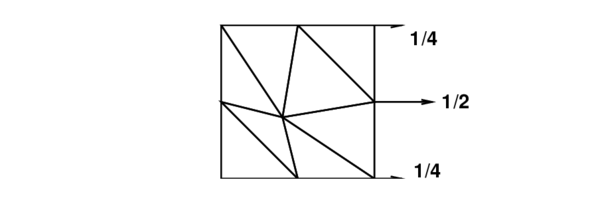Figure 4: Patch test for uniform tensile stress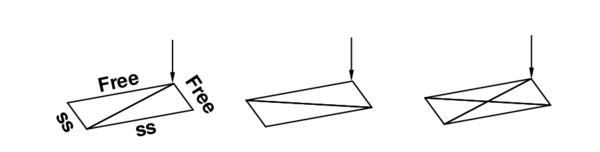Figure 5: Patch test for uniform torsion

The element bending formulation does not allow to apply external bending moments (there are not rotational DOFs). Hence it is not possible to analyse a patch of elements under loads leading to a uniform bending moment. A uniform torsion can be considered if a point load is applied at the corner of a rectangular plate with two consecutive free sides and two simple supported sides. Figure 5 shows three patches leading to correct results both in displacements and stresses. All three patches are structured meshes. When the central node in the third patch is shifted from the center, the results obtained with the EBST and EBST1 elements are not correct. This however does not seems to preclude the excellent performance of these elements, as proved in the rest of the examples analyzed. On the other hand, the BST element gives correct results in all torsion patch tests if natural boundary conditions are imposed in the formulation. If this is not the case, incorrect results are obtained even with structured meshes.

### 9.2 Cook's membrane problem

This example is used to assess the membrane performance of the EBST and EBST1 elements and to compare it with the standard linear triangle (constant strain) and the quadratic triangle (linear strain). This example involves important shear energy and was proposed to assess the distortion capability of elements. Figure 6.a shows the geometry and the applied load. Figure 6.b plots the vertical displacement of the upper vertex as a function of the number of nodes in the mesh. Results obtained with other isoparametric elements have also been plotted for comparison. They include the constant strain triangle (CST), the bilinear quadrilateral (QUAD4) and the linear strain triangle (LST) . Note that as this is a pure membrane problem the BST and the CST elements give identical results.

From the plot shown it can be seen that the enhanced element with three integration points (EBST) gives values slightly better that the constant strain triangle for the most coarse mesh (only two elements). However, when the mesh is refined a performance similar to the linear strain triangle is obtained that is dramatically superior than the former. On the other hand, if a one point quadrature is used (EBST1) the convergence in the reported displacement is notably better than for the rest of the elements.

### 9.3 Cylindrical roof

In this example an effective membrane interpolation is of primary importance. The geometry is a cylindrical roof supported by a rigid diaphragm at both ends and it is loaded by a uniform dead weight (see Figure 7.a.). Only one quarter of the structure is modelled due to symmetry conditions. Unstructured and structured meshes are considered. In the latter case two orientations are possible (Figure 7.a shows orientation B).

Tables 3, 4 and 5 present the normalized vertical displacements at the crown (point A) and at the midpoint of the free side (point B) for the two orientations of the structured meshes and for the non-structured mesh. Values used for normalization are ${\textstyle u_{A}=0.5407}$ y ${\textstyle u_{B}=-3.610}$ that are quoted in reference .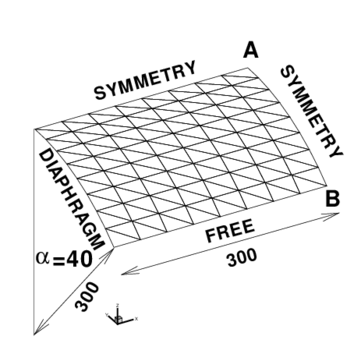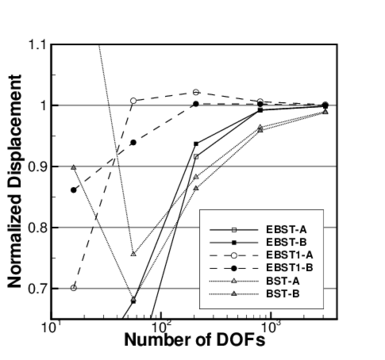Figure 7: Cylindrical roof under dead weight. $E=3\times 10^{6}$, $\nu =0.0$, Thickness =3.0, shell weight =0.625 per unit area. (a) Geometry and mesh for orientation B. (b) Displacement of point B for both (structured) mesh orientations

 Point-A Point-B NDOFs EBST EBST1 BST CBST EBST1 BST 16 0.65724 0.91855 0.74161 0.40950 0.70100 1.35230 56 0.53790 1.03331 0.74006 0.54859 1.00759 0.75590 208 0.89588 1.04374 0.88491 0.91612 1.02155 0.88269 800 0.99658 1.01391 0.96521 0.99263 1.00607 0.96393 3136 1.00142 1.00385 0.99105 0.99881 1.00102 0.98992

 Point-A Point-B NDOFs EBST EBST1 BST CBST EBST1 BST 16 0.26029 0.83917 0.40416 0.52601 0.86133 0.89778 56 0.81274 1.10368 0.61642 0.67898 0.93931 0.68238 208 0.97651 1.04256 0.85010 0.93704 1.00255 0.86366 800 1.00085 1.01195 0.95626 0.99194 1.00211 0.95864 3136 1.00129 1.00337 0.98879 0.99828 1.00017 0.98848

 Point-A Point-B NDOFs EBST EBST1 BST EBST EBST1 BST 851 0.97546 0.8581 0.97598 0.97662 1.0027 0.97194 3311 0.98729 0.9682 0.98968 0.98476 1.0083 0.98598 13536 0.99582 0.9992 1.00057 0.99316 0.9973 0.99596

Plots in Figure 7.b show the normalized displacement of point-B for structured meshes as a function of the number of degrees of freedom for each case studied. An excellent convergence for the EBST element can be seen. The version with only one integration point (EBST1) presents a behavior a little more flexible and converges from above for structured meshes. Table 5 shows that both the EBST and the EBST1 elements have an excellent behavior for non structured meshes.

### 9.4 Open semi-spherical dome with point loads

The main problem of shell finite elements with initially curved geometry is the so called membrane locking. The EBST element has a quadratic interpolation of the geometry, then it may suffer from this problem. To assess this we resort to an example of inextensional bending. This is an hemispherical shell of radius ${\textstyle r=10}$ and thickness ${\textstyle h=0.04}$ with an 18${\textstyle ^{o}}$ hole in the pole and free at all boundaries, subjected to two inward and two outward forces 90${\textstyle ^{o}}$ apart. Material properties are ${\textstyle E=6.825\times {10}^{7}}$ and ${\textstyle \nu =0.3}$. Figure 8.a shows the discretized geometry (only one quarter of the geometry is considered due to symmetry).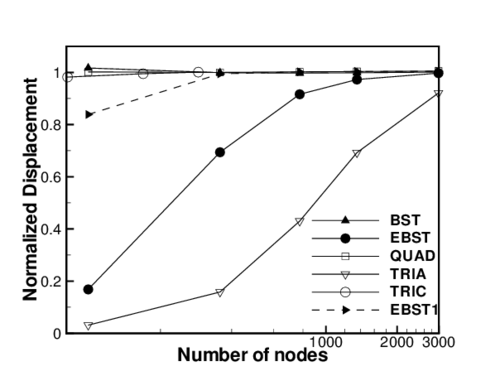Figure 8: Pinched hemispherical shell with a hole, (a)geometry, (b)normalized displacement

In Figure 8.b the displacements of the points under the loads have been plotted versus the number of nodes used in the discretization. Due to the orientation of the meshes chosen, the displacement of the point under the inward load is not the same as the displacement under the outward load, so in the figure an average (the absolute values) has been used. Results obtained with other elements have been included for comparison: three membrane locking free elements, namely the original linear BST element, a transverse shear-deformable quadrilateral (QUAD)  and an assumed strain quadratic triangle (TRIC) ; a transverse shear deformable quadratic triangle (TRIA) (standard displacement formulation for membrane part)  that is vulnerable to locking.

From the plotted results it can be seen that the EBST element presents slight membrane locking in bending dominated problems with initially curved geometries. This locking is much less severe than in a standard quadratic triangle. Membrane locking disappears when only one integration point is used (EBST1 element).

### 9.5 Inflation of a sphere

The example is the inflation of a spherical shell under internal pressure. An incompressible Mooney-Rivlin constitutive material has been considered. The Ogden parameters are ${\textstyle N=2}$, ${\textstyle \alpha _{1}=2}$, ${\textstyle \mu _{1}=40}$, ${\textstyle \alpha _{2}=-2}$, ${\textstyle \mu _{2}=-20}$. Due to the simple geometry an analytical solution exists  (with ${\textstyle \gamma =R/R^{0}}$):

 $p={\frac {h^{0}}{R^{0}\gamma ^{2}}}{\frac {dW}{d\gamma }}={\frac {8h^{0}}{R^{0}\gamma ^{2}}}\left(\gamma ^{6}-1\right)\left(\mu _{1}-\mu _{2}\gamma ^{2}\right)$

In this numerical simulation the same geometric and material parameters used in  have been adopted: ${\textstyle R^{0}=1}$ and ${\textstyle h^{0}=0.02}$. The three meshes of EBST1 element considered to evaluate convergence are shown in Figure 9.a. The value of the actual radius as a function of the internal pressure is plotted in Figure 9.b for the different meshes and is also compared with the analytical solution. It can be seen that with a few degrees of freedom it is possible to obtain an excellent agreement for the range of strains considered. The final value corresponds to a ratio of ${\textstyle h/R=0.00024}$.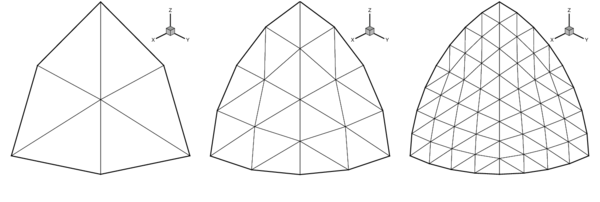(a)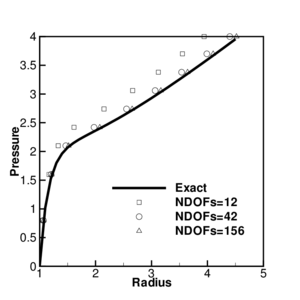(b) Figure 9: Inflation of sphere of Mooney-Rivlin material. (a) Meshes of EBST1 elements used in the analysis. (b) Radius as a function of the internal pressure.

The geometry of the dome and the material properties chosen are shown in Figure 10. A uniform pressure load of 600 psi is applied to the upper surface of the dome. The different meshes used in the analysis are shown in Figure 11. One fourth of the dome is considered only due to symmetry. Two different analyses under elastic and elastic-plastic conditions were carried out. The number of thickness layers in eq.(100) is four. Numerical experiments show that this suffices to provide an accurate solution for large elastic-plastic problems . Results are obtained using the explicit scheme.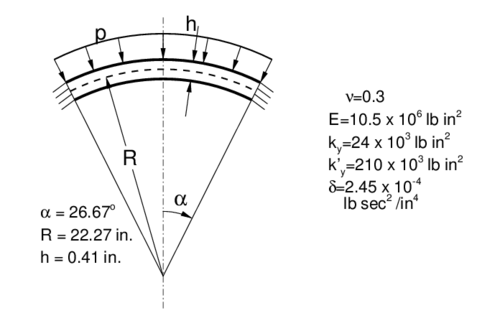Figure 10: Spherical dome under impulse pressure. Geometry and material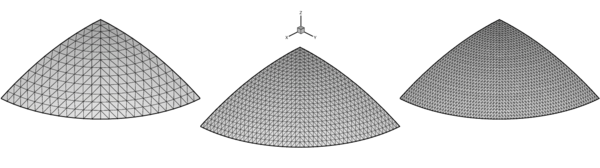Figure 11: Spherical dome under impulse pressure. Meshes used in the analysis. Mesh-1 with 338 elements, Mesh-2 with 1250 elements and Mesh-3 with 2888 elements

Figure 12 shows results for the time history of the central deflection using different meshes and elastic material properties for both BST and EBST1 elements. Results are almost identical for mesh-2 and mesh-3, showing the excellent convergence properties. The coarsest mesh shows some differences between both elements, but for the finer meshes the results are almost identical. Figure 13 shows similar results but now for an elastic-plastic material. The excellent convergence of the BST and EBST1 elements is again noticeable.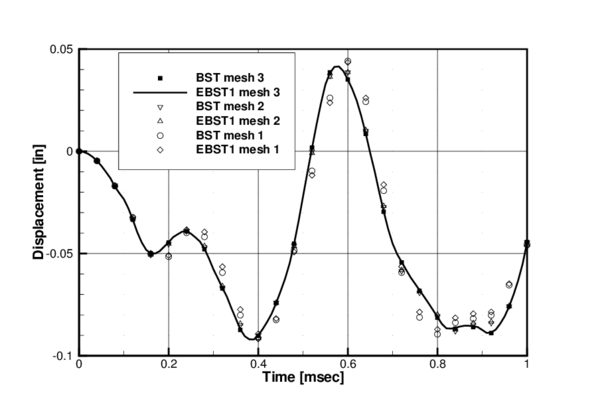Figure 12: Spherical dome under impulse pressure. History of central deflection for elastic material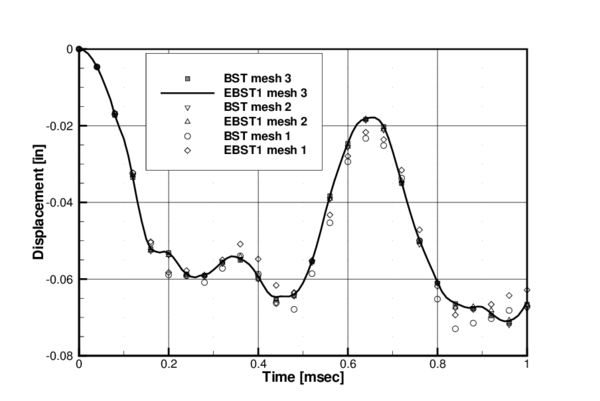Figure 13: Spherical dome under impulse pressure. History of central deflection for elastic-plastic material

Results obtained with the present elements compare very well with published results using fine meshes. See for example ABAQUS Explicit example problems manual  and WHAMS-3D manual , showing plots comparing results using different shell elements.

A summary of results for the central deflection at significant times is given in Tables 6 and 7. Further details on the solution of this problem with the standard BST element can be found in .

 Element/mesh $t=0.2ms$ $t=0.4ms$ $t=0.6ms$ $t=0.8ms$ BST Coarse -0.05155 -0.09130 0.04414 -0.08945 BST Medium -0.04542 -0.09177 0.03863 -0.08052 BST Fine -0.04460 -0.09022 0.03514 -0.08132 EBST1 Coarse -0.05088 -0.08929 0.04348 -0.08708 EBST1 Medium -0.04527 -0.09134 0.03865 -0.07979 EBST1 Fine -0.04453 -0.09004 0.03510 -0.08099

 Element/mesh $t=0.2ms$ $t=0.4ms$ $t=0.6ms$ $t=0.8ms$ BST Coarse -0.05888 -0.05869 -0.02938 -0.06521 BST Medium -0.05376 -0.06000 -0.02564 -0.06098 BST Fine -0.05312 -0.05993 -0.02464 -0.06105 EBST1 Coarse -0.05827 -0.05478 -0.02792 -0.06187 EBST1 Medium -0.05374 -0.05884 -0.02543 -0.06080 EBST1 Fine -0.05317 -0.05935 -0.02458 -0.06085

The geometry of the cylinder and the material properties are shown in Figure 14. A prescribed initial normal velocity of ${\textstyle v_{o}=-5650}$ in/sec is applied to the points in the region shown modelling the effect of the detonation of an explosive layer. The panel is assumed clamped along all the boundary. One half of the cylinder is discretized only due to symmetry conditions. Three different meshes of ${\textstyle 6\times {12}}$, ${\textstyle 12\times {32}}$ and ${\textstyle 18\times {48}}$ triangles were used for the analysis. The deformed configurations for ${\textstyle time=1msec}$ are shown for the three meshes in Figure 15.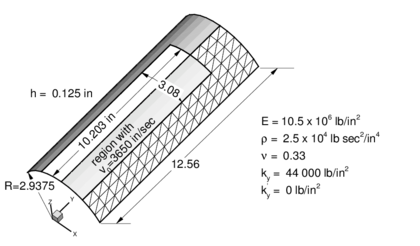Figure 14: Cylindrical panel under impulse loading. Geometry and material properties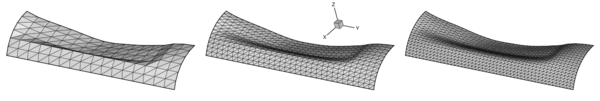Figure 15: Impulsively loaded cylindrical panel. Deformed meshes for $time=1msec$

The analysis was performed assuming an elastic-perfect plastic material behaviour (${\textstyle \sigma _{y}=k_{y}}$ ${\textstyle k_{y}'=0}$). A study of the convergence of the solution with the number of thickness layers showed again that four layers suffice to capture accurately the non linear material response .

A comparison of the results obtained with the BST and EBST1 elements using the coarse mesh and the finer mesh is shown in Figure 16 where experimental results reported in  have also been plotted for comparison purposes. Good agreement between the numerical and experimental results is obtained. Figures 16 show the time evolution of the vertical displacement of two reference points along the center line located at ${\textstyle y=6.28}$in and ${\textstyle y=9.42}$in, respectively. For the finer mesh results between both elements are almost identical. For the coarse mesh it can been seen again that the element BST is more flexible than element EBST1.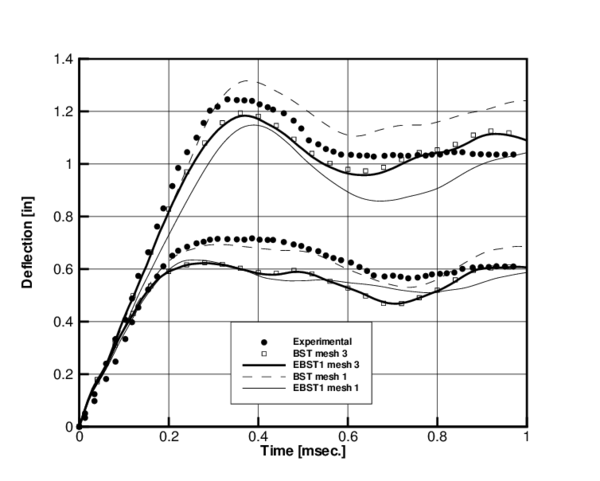Figure 16: Cylindrical panel under impulse loading. Time evolution of the displacement of two points along the crown line. Comparison of results obtained with BST and EBST1 elements (mesh 1: $6\times {12}$ elements and mesh 3: $18\times {48}$ elements) and experimental values

The numerical values of the vertical displacement at the two reference points obtained with the BST and EBST1 elements after a time of 0.4 ms using the ${\textstyle 16\times {32}}$ mesh are compared in Table 8 with a numerical solution obtained by Stolarski et al.  using a curved triangular shell element and the ${\textstyle 16\times {32}}$ mesh. Experimental results reported in  are also given for comparison. It is interesting to note the reasonable agreement of the results for ${\textstyle y=6.28}$in. and the discrepancy of present and other published numerical solutions with the experimental value for ${\textstyle y=9.42}$in.

 Vertical displacement (in.) element/mesh $y=6.28$in $y=9.42$in BST (${\textstyle 6\times 12}$ el.) -1.310 -0.679 BST (${\textstyle 18\times 48}$ el.) -1.181 -0.587 EBST1 (${\textstyle 6\times 12}$ el.) -1.147 -0.575 EBST1 (${\textstyle 18\times 48}$ el.) -1.171 -0.584 Stolarski et al.  -1.183 -0.530 Experimental  -1.280 -0.700

The deformed shapes of the transverse section for ${\textstyle y=6.28}$in. and the longitudinal section for ${\textstyle x=0}$ obtained with the both elements for the coarse and the fine meshes after 1ms. are compared with the experimental results in Figures 17 and 18. Excellent agreement is observed for the fine mesh for both elements.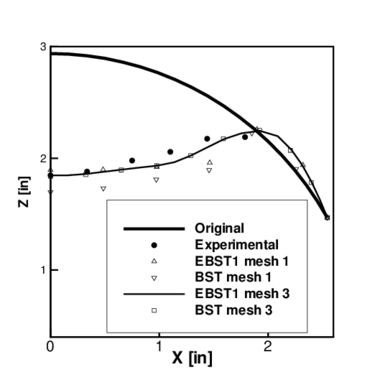Figure 17: Cylindrical panel under impulse loading. Final deformation ($t=1msec$) of the panel at the cross section $y=6.28in$ Comparison with experimental values.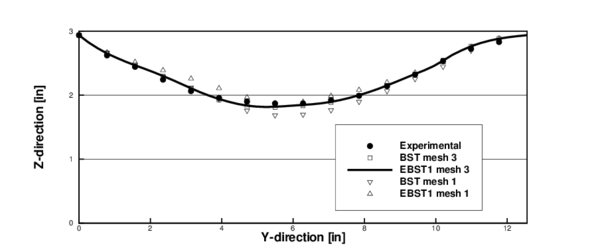Figure 18: Cylindrical panel under impulse loading. Final deformation ($t=1msec$) of the panel at the crown line ($x=0.00in$). Comparison with experimental values.

### 9.8 Airbag Membranes

Inflation/deflation of a circular airbag

This example has been taken from Ref. where it is shown that the final configuration is mesh dependent due to the strong instabilities leading to a non-uniqueness of the solution. In  it is also discussed the important regularizing properties of the bending energy that, when disregarded, leads to massive wrinkling in the compressed zones.

The airbag geometry is initially circular with an undeformed radius of ${\textstyle 0.35}$. The material is a linear isotropic elastic one with modulus of elasticity ${\textstyle E=6\times 10^{7}}$Pa, Poisson's ratio ${\textstyle \nu =0.3}$ and density ${\textstyle \rho =2000}$kg/m${\textstyle ^{3}}$. A symmetrical solution has been assumed and, hence, only one quarter of the geometry has been modelled. Only the normal displacement to the original plane is constrained along the boundaries. The thickness considered is ${\textstyle h=0.0004}$m and the inflation pressure is ${\textstyle 5000}$Pa. Pressure is linearly increased from 0 to the final value in ${\textstyle t=0.15}$ sec.

Figure 19 shows the final deformed configurations for a mesh with 10201 nodes and 20000 EBST1 elements. The figure on the left (a) corresponds to an analysis including full bending effects and the right figure (b) is a pure membrane analysis.

We note that when the bending energy is included a more regular final pattern is obtained. Also the final pattern is rather independent of the discretization (note that the solution is non unique due to the strong instabilities). On the other hand, the pure membrane solution shows in the center of the modelled region a wrinkling pattern where the width of the wrinkle is the length of the element.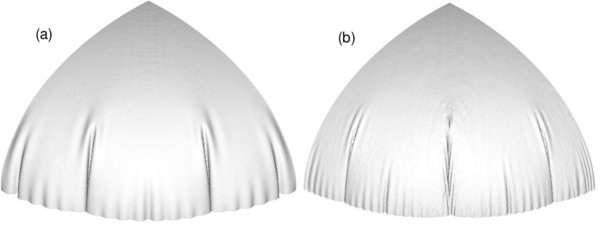Figure 19: Inflation of a circular airbag. Deformed configurations for final pressure. (a) bending formulation; (b) membrane formulation.

Figure 20 shows the results obtained for the de-inflation process. Note that the spherical membrane falls down due to the self weight. The configurations are, of course, non-unique.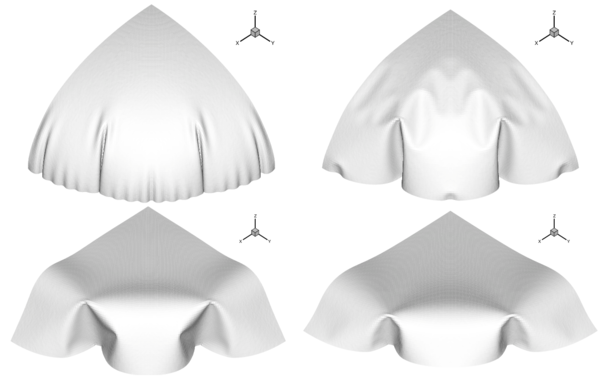Figure 20: Inflation and deflation of a circular air-bag.

### Inflation/deflation of a closed tube

The next problem is the study of the inflating and de-inflating of a tube with a semi-spherical end cap. The tube diameter is ${\textstyle D=2}$m, its total length is ${\textstyle L=5}$m and the thickness ${\textstyle h=5\times 10^{-3}}$m. The material has the following properties: ${\textstyle E=4\times 10^{8}}$Pa, ${\textstyle \nu =0.35}$ and ${\textstyle \rho =3\times 10^{3}}$kg/m${\textstyle ^{3}}$. The tube is inflated fast until a pressure of ${\textstyle 10^{4}}$Pa and then is de-inflated under self weight. The analysis is performed with a mesh of 4176 EBST1 elements and 2163 nodes modelling a quarter of the geometry. The evolution of the tube walls during the de-inflating process can be seen in Figure 21. Note that the central part collapses as expected, while the semi-spherical cap remains rather unaltered.

The same analysis is repeated for a longer and thinner tube (${\textstyle L=6}$m and ${\textstyle h=3\times 10^{-3}}$m). The same material than in the previous case was chosen. The evolution of the tube walls is shown in Figure 22. Note that the central part collapses again but in a less smoother manner due to the smaller thickness.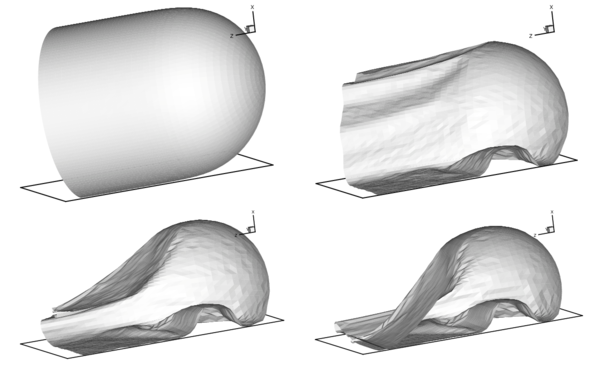Figure 21: Inflation and deflation of a closed tube. $L=5$, $D=2$, $h=5\times 10^{-4}$.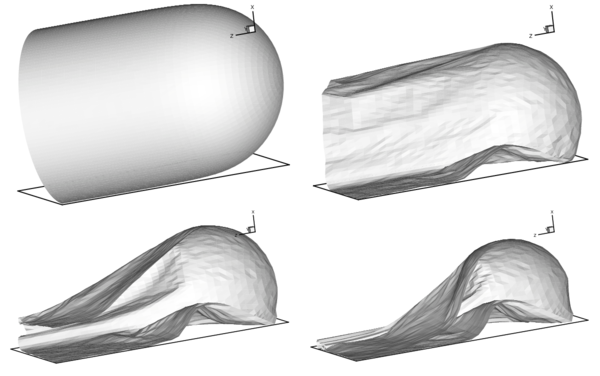Figure 22: Inflation and deflation of a closed tube. $L=6$, $D=2$, $h=3\times 10^{-4}$.

Inflation of a square airbag

The last example of this kind is the inflation of a square airbag supporting a spherical object. This example resembles a problem studied (numerically and experimentally) in , where fluid-structure interaction is the main subject. Here the fluid is not modelled and a uniform pressure is applied over all the internal surfaces. The lower surface part of the airbag is limited by a rigid plane and on the upper part a spherical dummy object is set to study the interaction between the airbag and the object.

The airbag geometry is initially square with an undeformed side length of 0.643m. The constitutive material chosen is a linear isotropic elastic one with ${\textstyle E=5.88\times 10^{8}}$Pa, ${\textstyle \nu =0.4}$ and a density of ${\textstyle \rho =1000}$ kg/m${\textstyle ^{3}}$. Only one quarter of the geometry has been modelled due to symmetry. The thickness ${\textstyle h=0.00075}$m and the inflation pressure is 250000Pa. Pressure is linearly incremented from 0 to the final value in ${\textstyle t=0.15}$sec. The spherical object has a radius ${\textstyle r=0.08}$m and a mass of 4.8kg (one quarter) and is subjected to gravity load during all the process.

The mesh has 8192 EBST1 elements and 4225 nodes on each surface of the airbag. Figure 23 shows the deformed configurations for three different times. The sequence on the left of the figure corresponds to an analysis including full bending effects and that on the right is the result of a pure membrane analysis. A standard penalty formulation is used in order to treat the frictionless contact.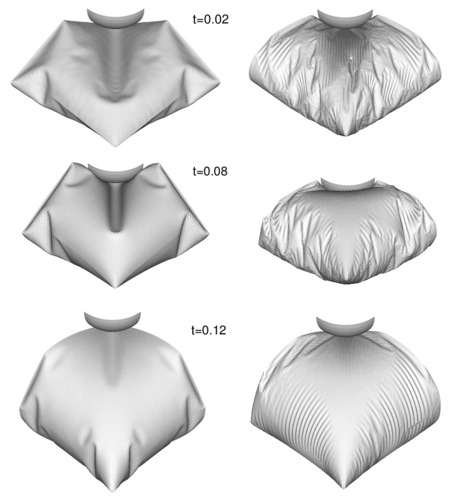Figure 23: Inflation of a square airbag against an spherical object. Deformed configurations for different times. Left figure: results obtained with the full bending formulation. Right figure: results obtained with a pure membrane solution.

### 9.9 S-rail sheet stamping

The final problem corresponds to one of the sheet stamping benchmark tests proposed in NUMISHEET'96 . The analysis comprises two parts, namely, simulation of the stamping of a S-rail sheet component and springback computations once the stamping tools are removed. Figure 24 shows the deformed sheet after springback.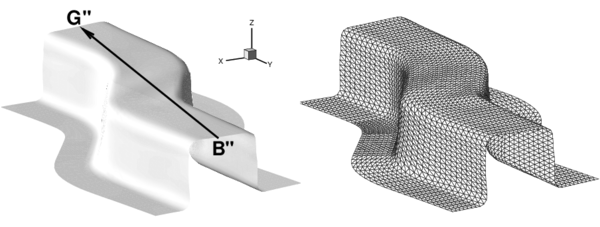Figure 24: Stamping of a S-rail. Final deformation of the sheet after springback obtained in the simulation. The triangular mesh of the deformed sheet is also shown

The detailed geometry and material data can be found in the proceedings of the conference  or in the web . The mesh used for the sheet has 6000 three node triangular elements and 3111 points (Figure 24). The tools are treated as rigid bodies. The meshes used for the sheet and the tools are those provided by the benchmark organizers. The material considered here is a mild steel (IF) with Young Modulus ${\textstyle E=2.06GPa}$ and Poisson ratio ${\textstyle \nu =0.3}$. Mises yield criterion was used for plasticity behaviour with non-linear isotropic hardening defined by ${\textstyle \sigma _{y}(e^{p})=545(0.13+e^{p})^{0.267}[MPa]}$. A uniform friction of 0.15 was used for all the tools. A low (10kN) blank holder force was considered in this simulation.

Figure 25 compares the punch force during the stamping stage obtained with both BST and EBST1 elements for the simulation and experimental values. Also for reference the average values of the simulations presented in the conference are included. Explicit and implicit simulations are considered as different curves. There is a remarkable coincidence between the experimental values and the results obtained with BST and EBST1 elements.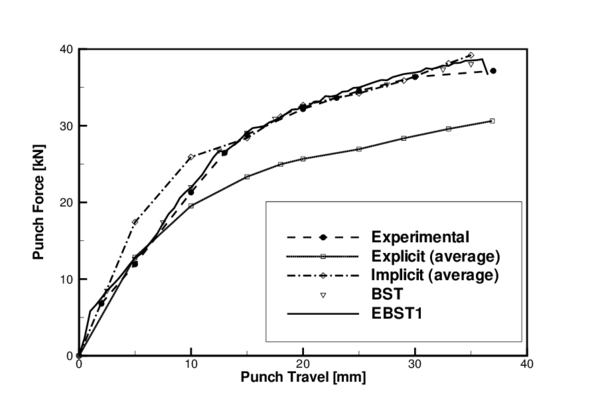Figure 25: Stamping of a S-rail. Punch force versus punch travel. Average of explicit and implicit results reported at the benchmark are also shown.

Figure 26 plots the ${\textstyle Z}$ coordinate along line B"–G" after springback. The top surface of the sheet does not remain plane due to some instabilities due to the low blank holder force used. Results obtained with the simulations compare very well with the experimental values.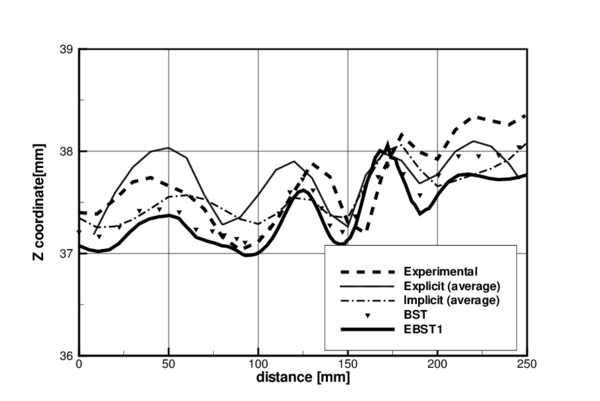Figure 26: Stamping of a S-rail. Z-coordinate along line B–-G after springback. Average of explicit and implicit results reported at the benchmark are also shown.

## 10 CONCLUDING REMARKS

We have presented in the paper two alternative formulations for the rotation-free basic shell triangle (BST) using an assumed strain approach. The simplest element of the family is based on an assumed constant curvature field expressed in terms of the nodal deflections of a patch of four elements and a constant membrane field computed from the standard linear interpolation of the displacements within each triangle. An enhanced version of the BST element is obtained by using a quadratic interpolation of the geometry in terms of the six patch nodes. This allows to compute an assumed linear membrane strain field which improves the in-plane behaviour of the original element. A simple and economic version of the new EBST element using a single integration point has been presented. The efficiency of the different rotation-free shell triangles has been demonstrated in many examples of application including linear and non linear analysis of shells under static and dynamic loads, the inflation and de-inflation of membranes and a sheet stamping problem.

The enhanced rotation-free basic shell triangle element with a single integration point (the EBST1 element) has proven to be an excellent candidate for solving practical engineering shell and membrane problems involving complex geometry, dynamics, material non linearity and frictional contact conditions.

## ACKNOWLEDGEMENTS

The problems analyzed with the explicit formulation were solved with the computer code STAMPACK  where the rotation-free elements here presented have been implemented. The support of the company QUANTECH (www.quantech.es) providing the code STAMPACK is gratefully acknowledged.